# RD Sharma Solutions for Class 7 Maths Chapter 14 Lines And Angles

Get free PDF of RD Sharma Solutions for Class 7 Maths Chapter 14 Lines and Angles from the given links. Students who aim to top the examinations can refer to RD Sharma Solutions. BYJU’S expert team has solved the questions in such a way that learners can understand easily and comfortably. Learners can simply download the PDFs from the available links.

Chapter 14, Lines And Angles includes two exercises. RD Sharma Solutions for Class 7 includes answers to all questions present in these exercises. Let us have a look at some of the concepts that are being discussed in this Chapter.

• Pairs of Angles
• Definition and meaning of adjacent angles
• Definition and meaning of linear pair
• Vertically opposite angles
• Definition of angles at a point
• Concept of complementary angles
• Concept of supplementary angles
• Definition and meaning of parallel lines
• Parallel rays
• Parallel segments
• Transversals
• Angle made by a transversal with two lines
• Exterior and interior angles
• Corresponding angles
• Alternate interior and interior angles
• Angles made by a transversal to two parallel lines

## Download the PDF of RD Sharma Solutions For Class 7 Maths Chapter 14 Lines And Angles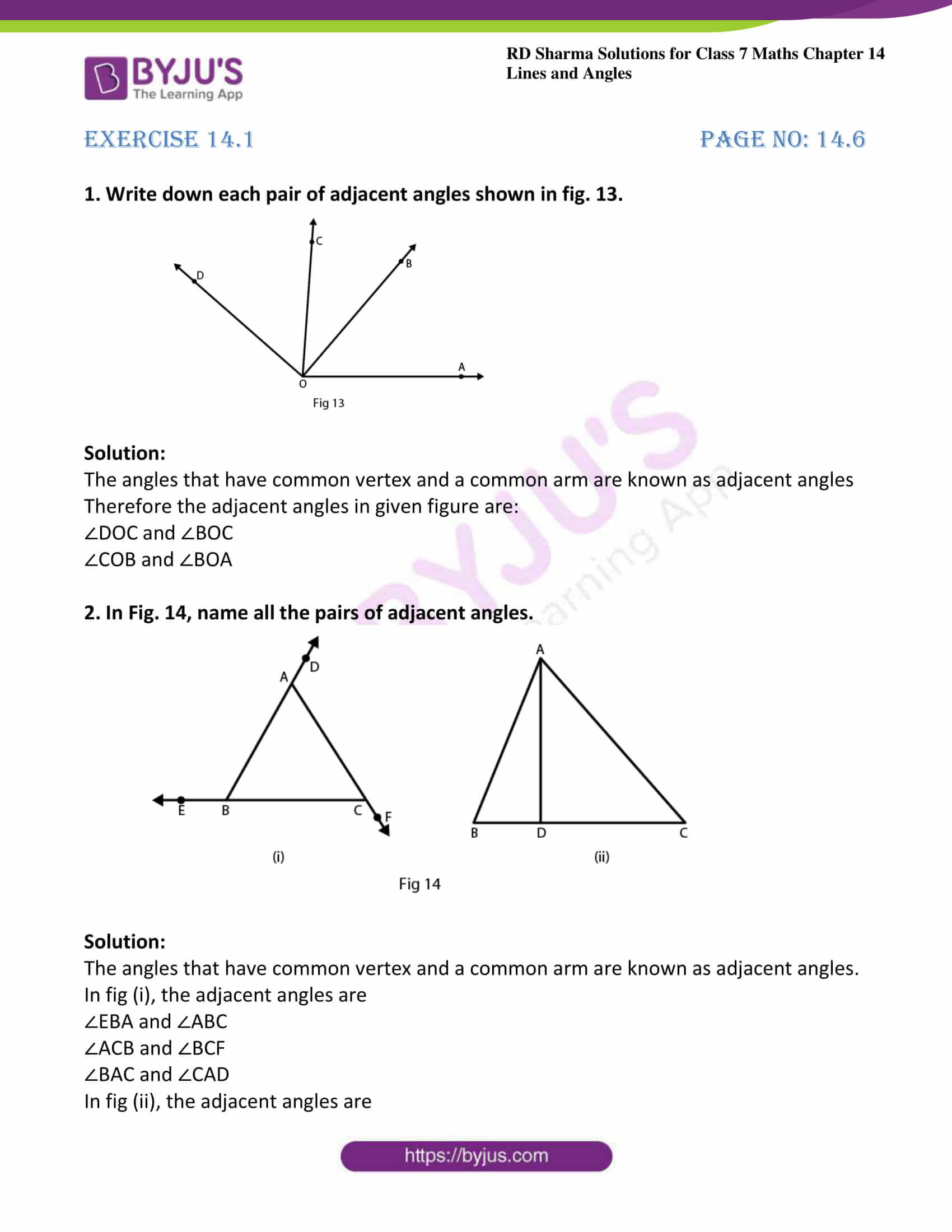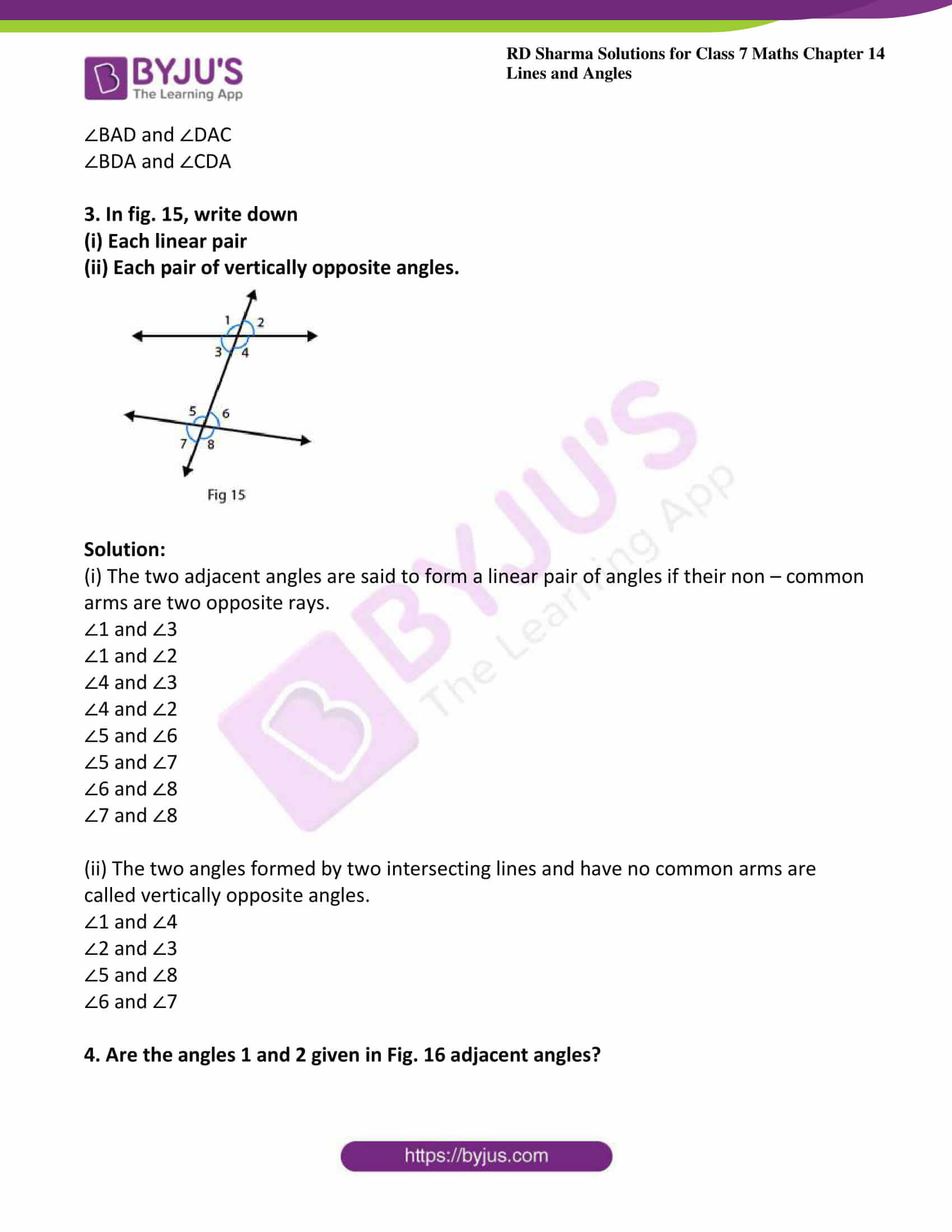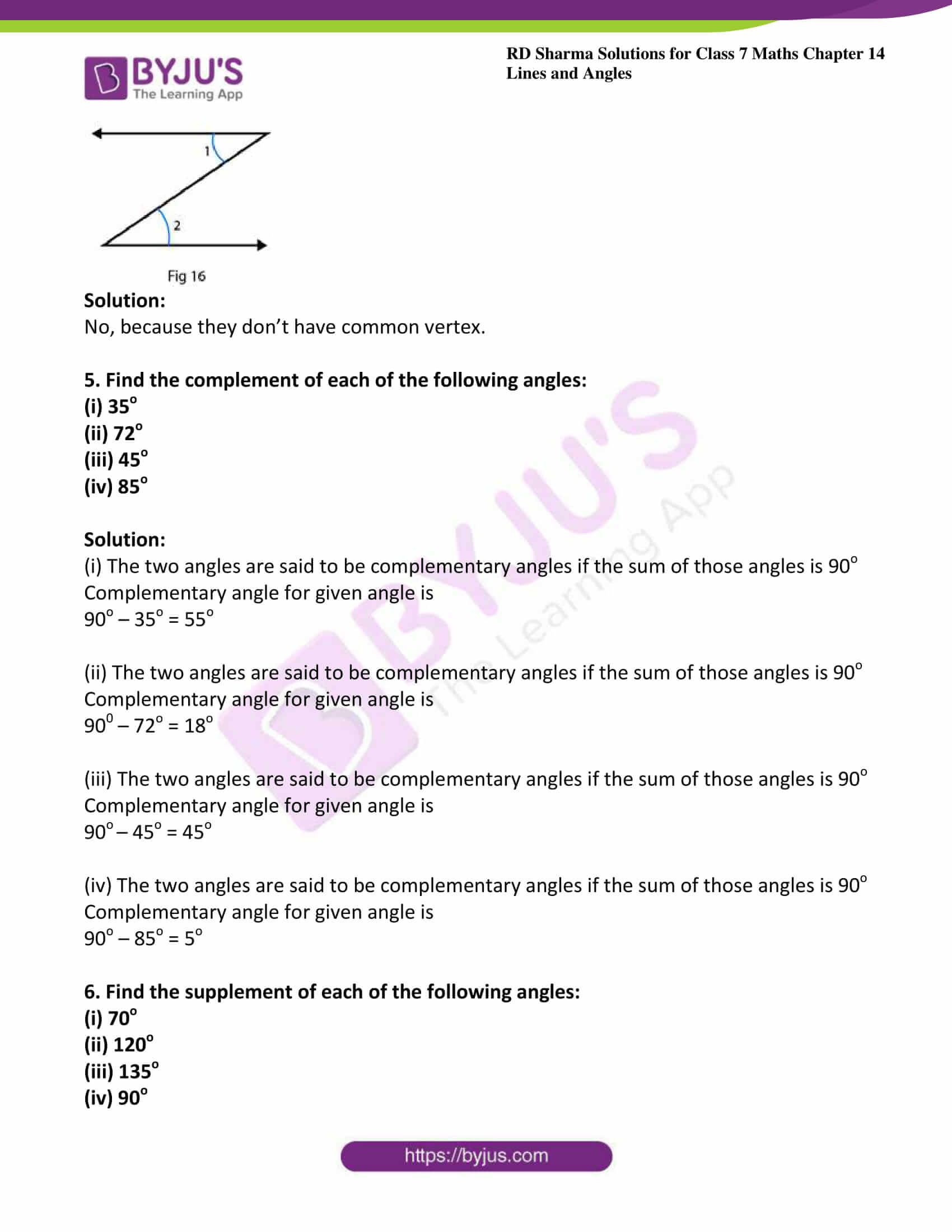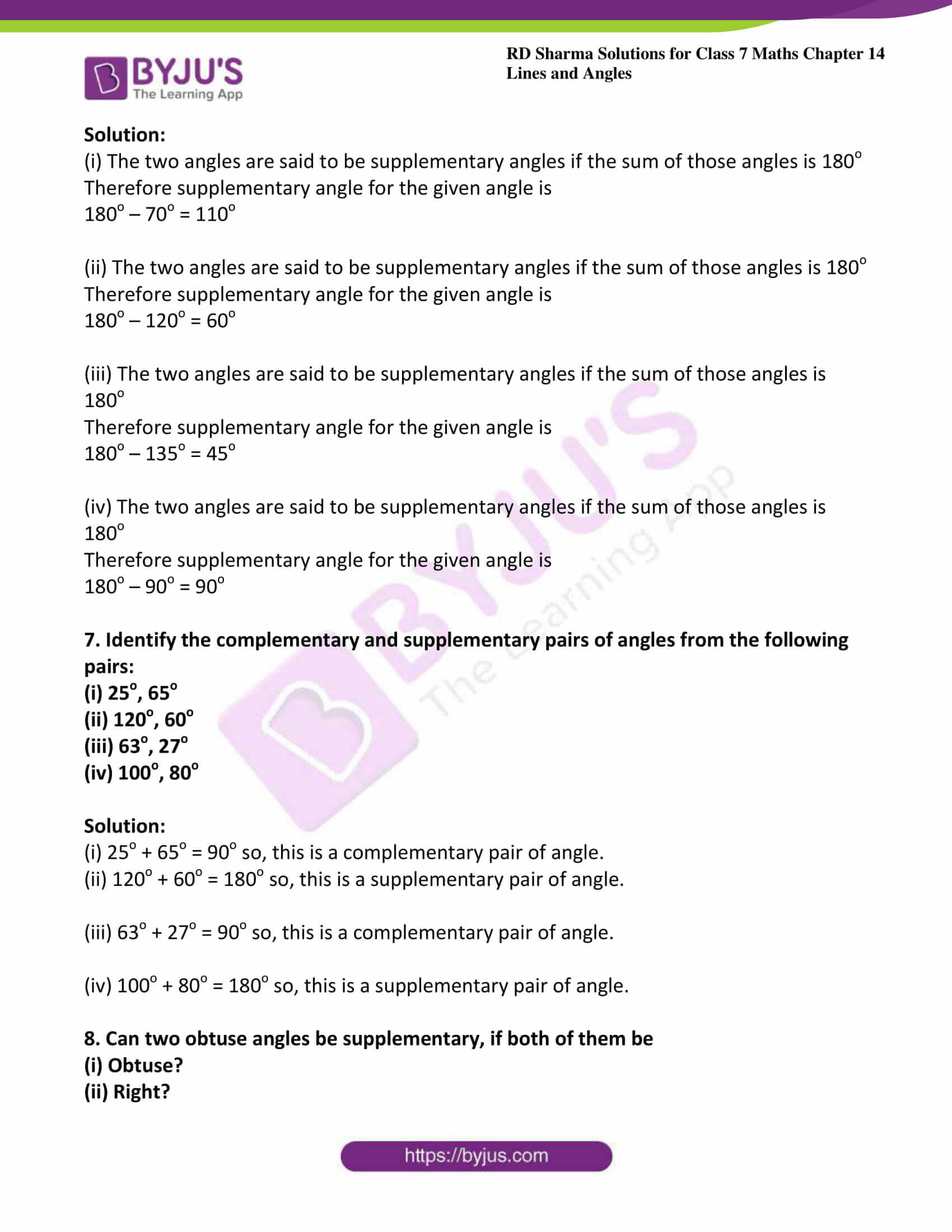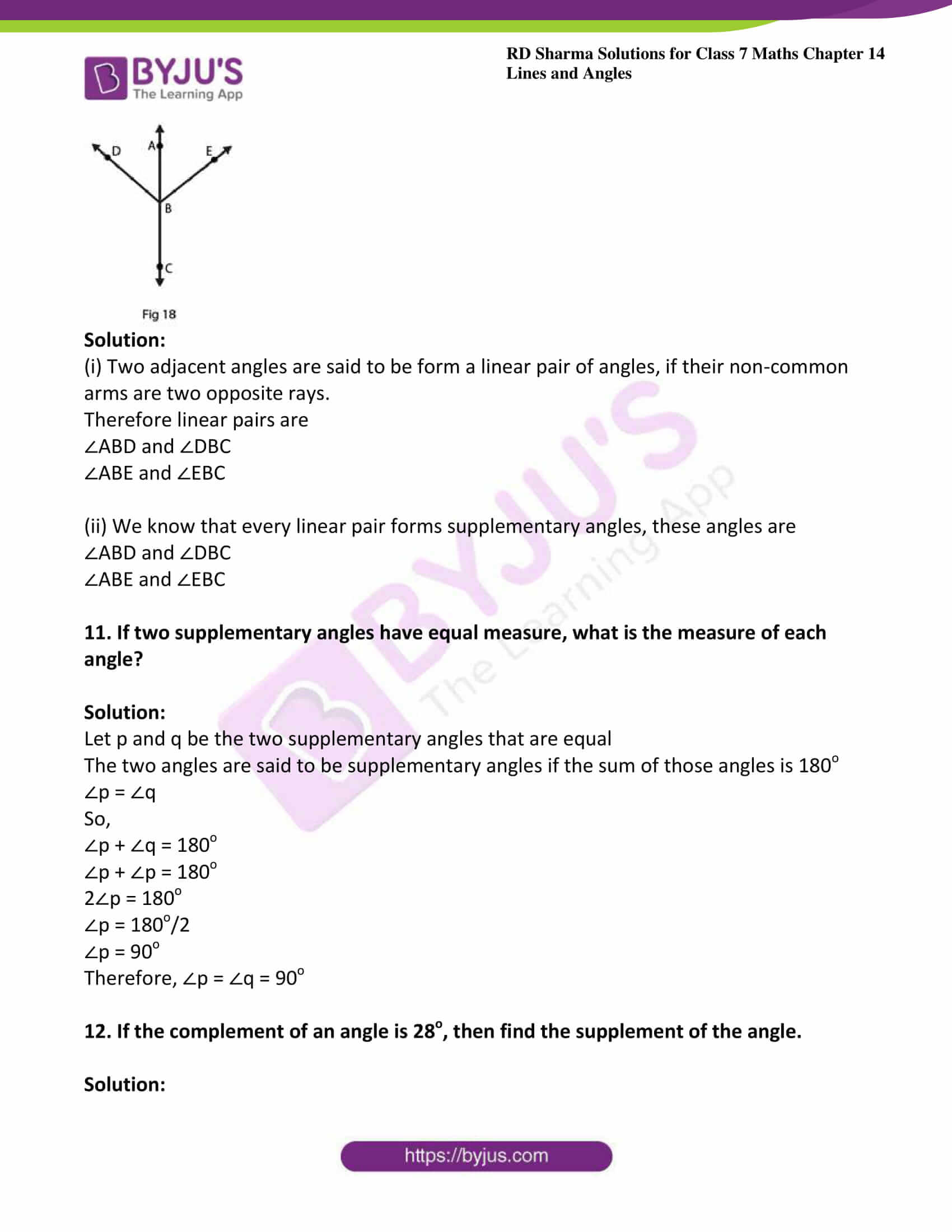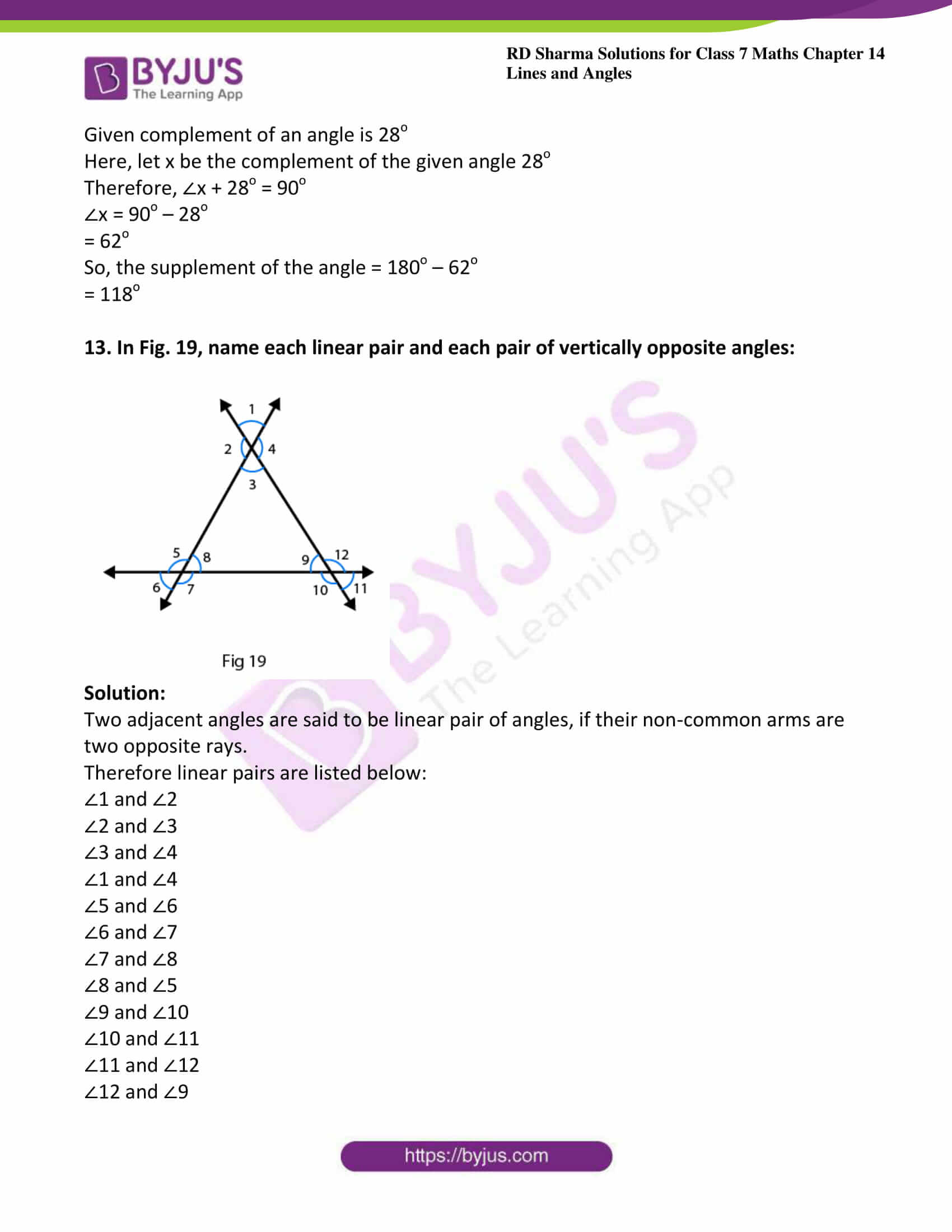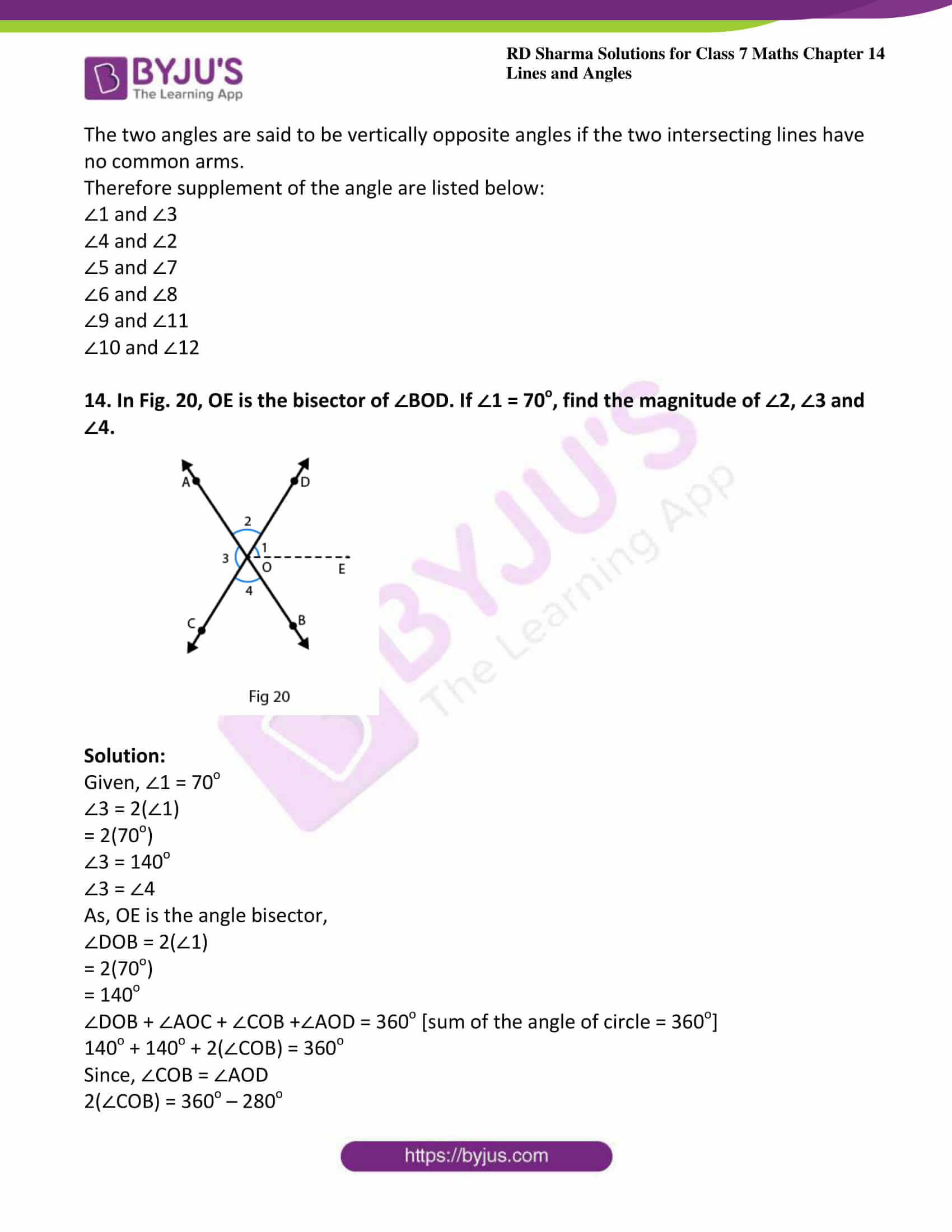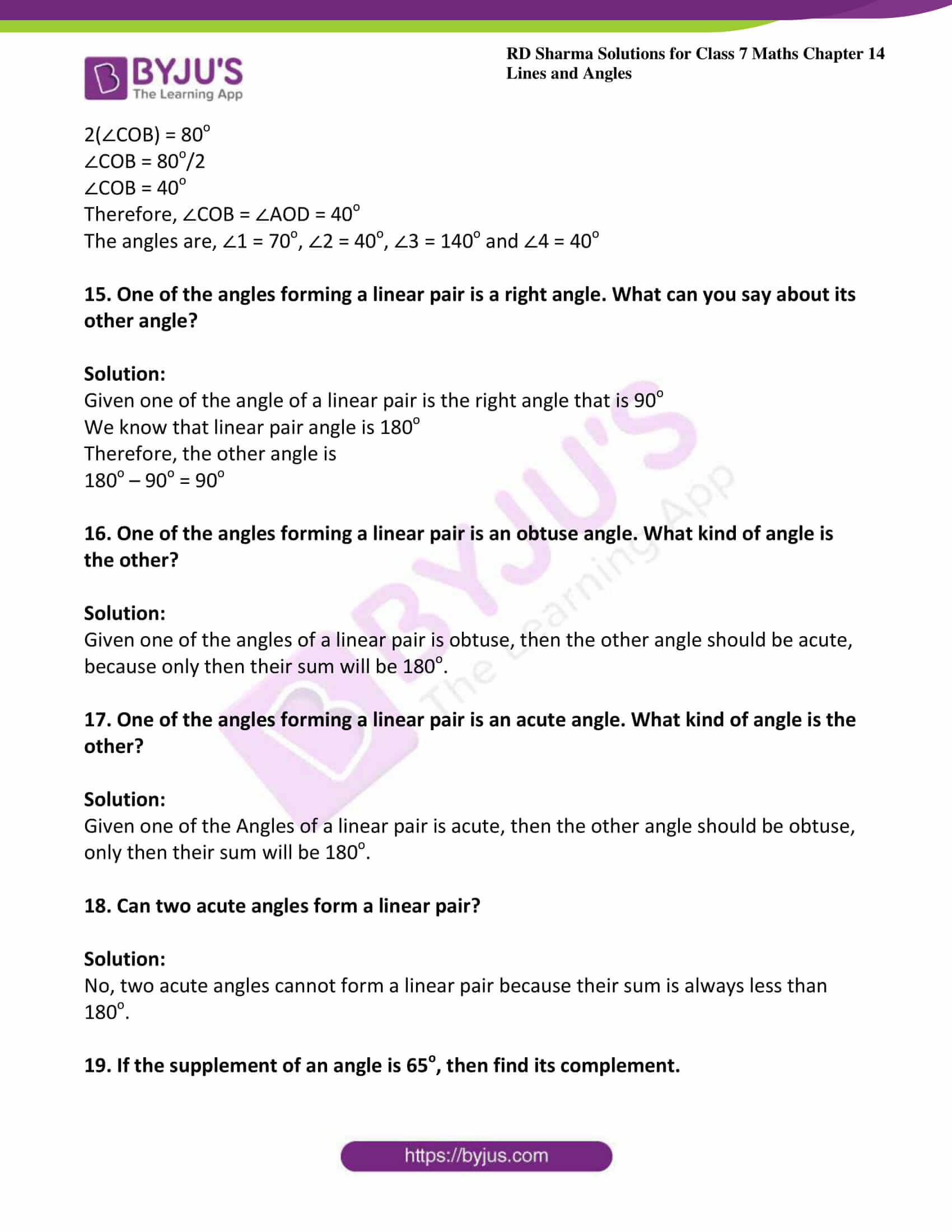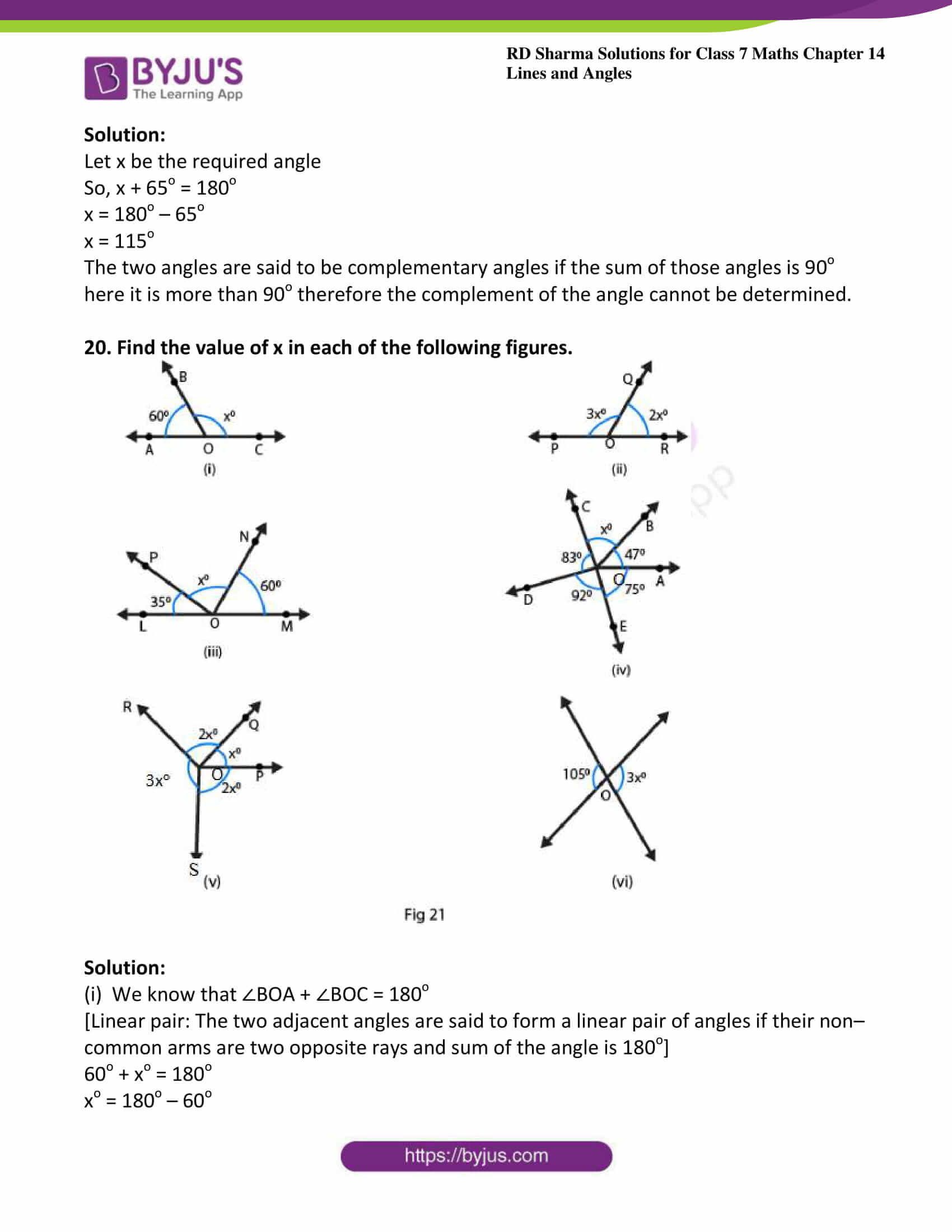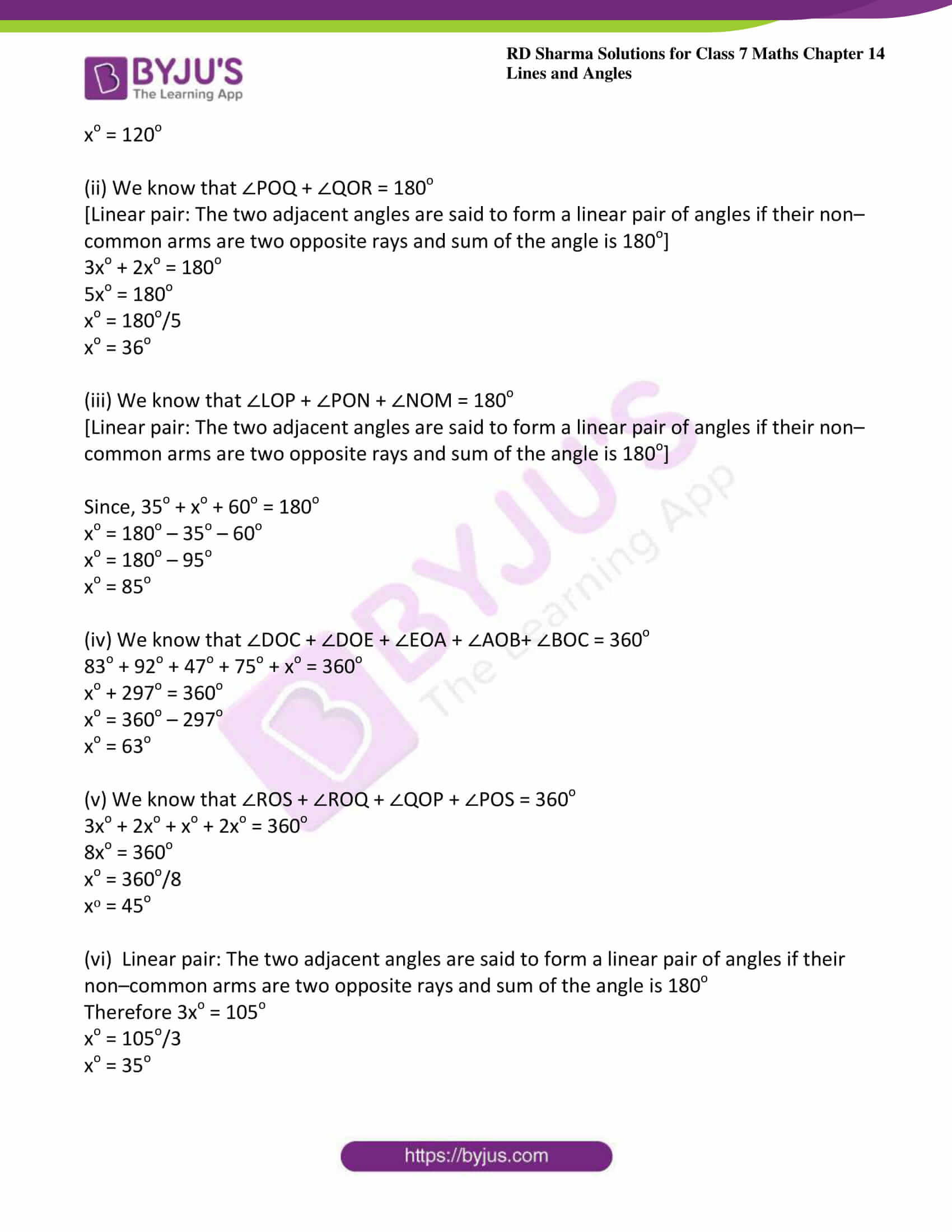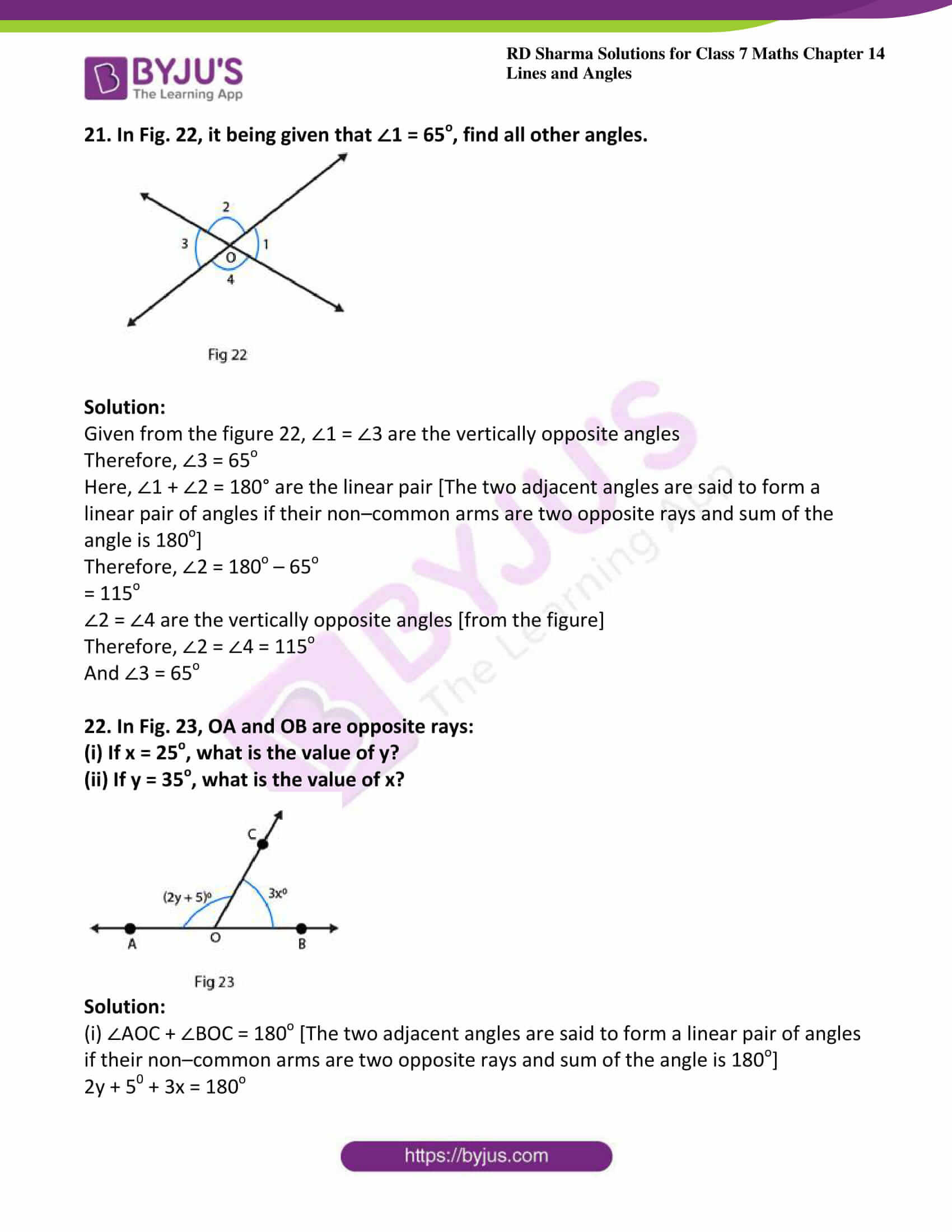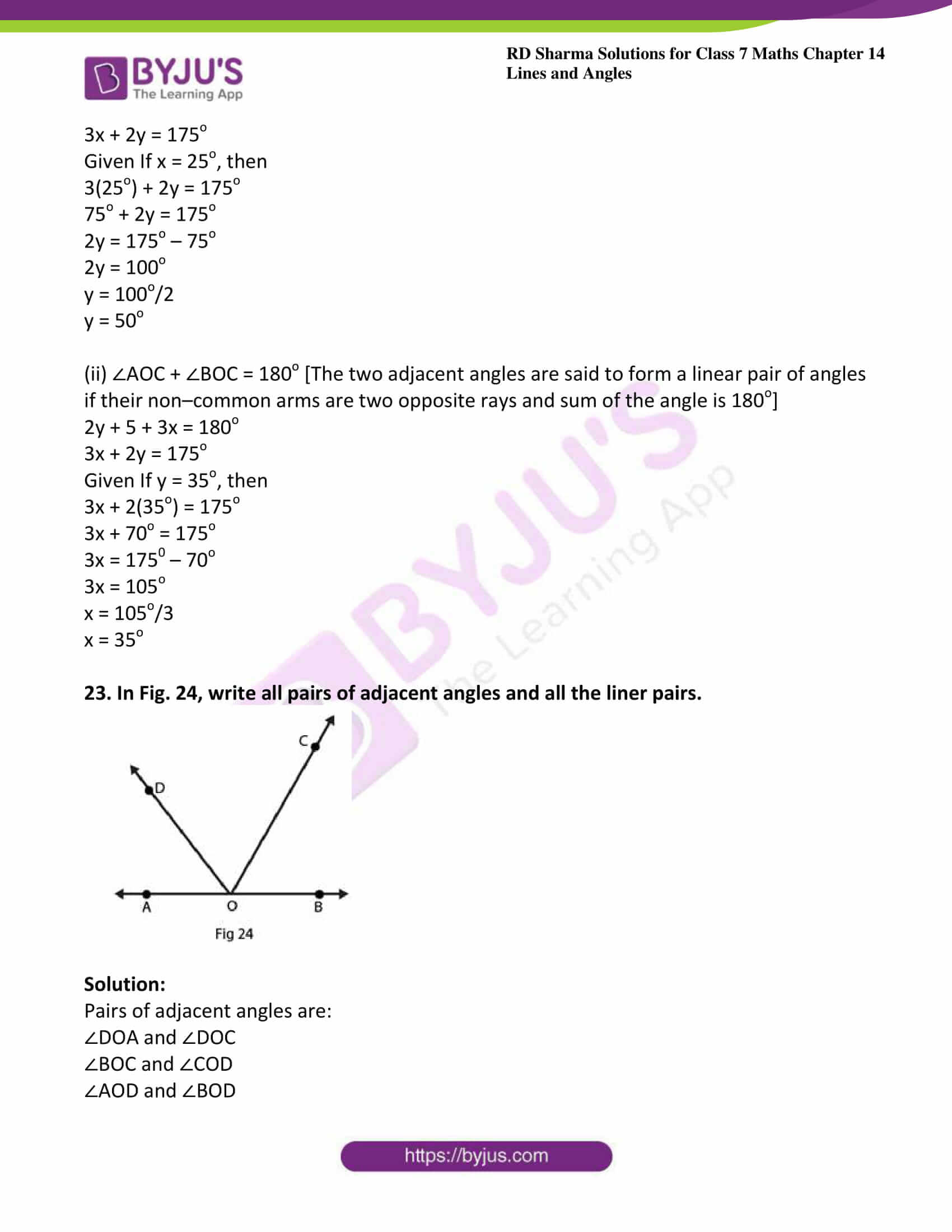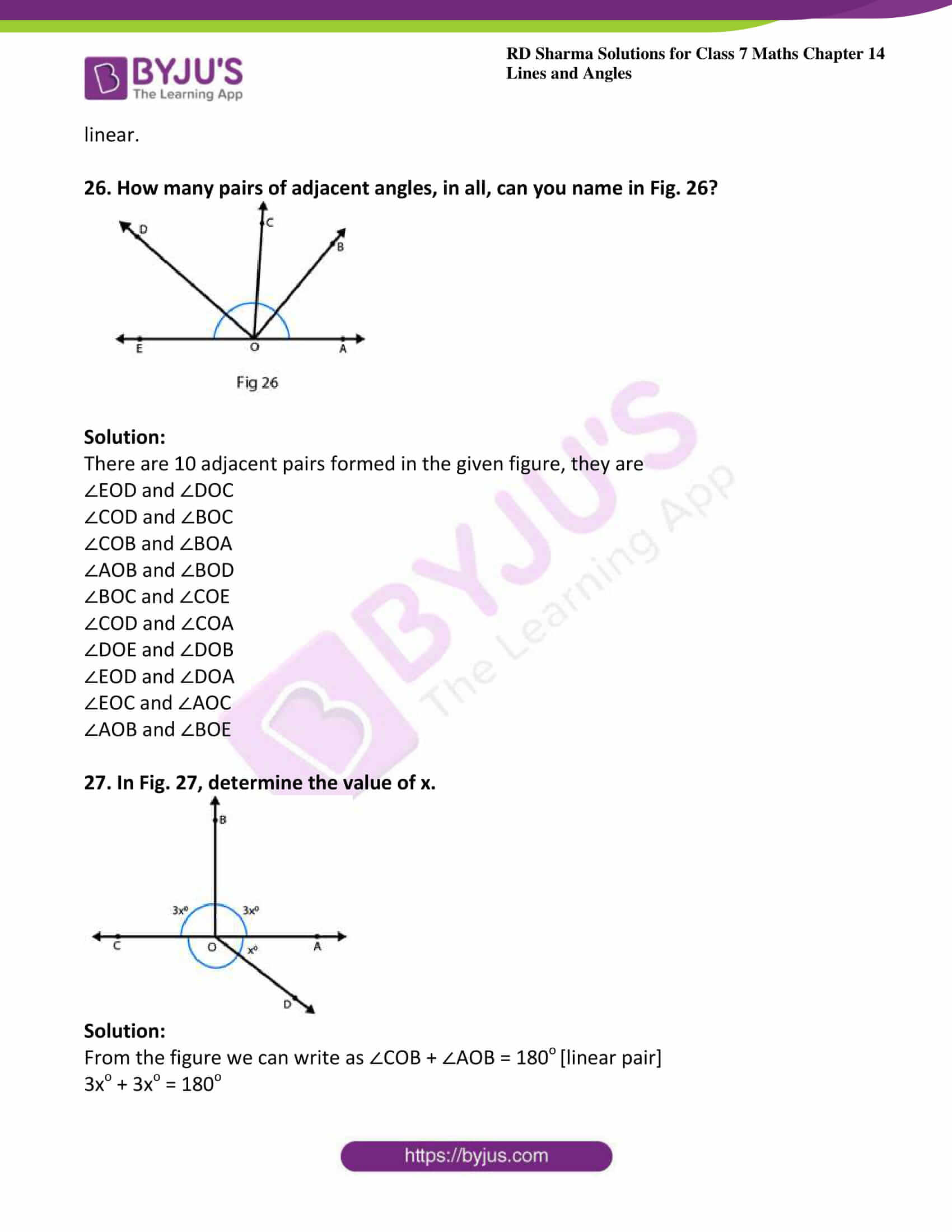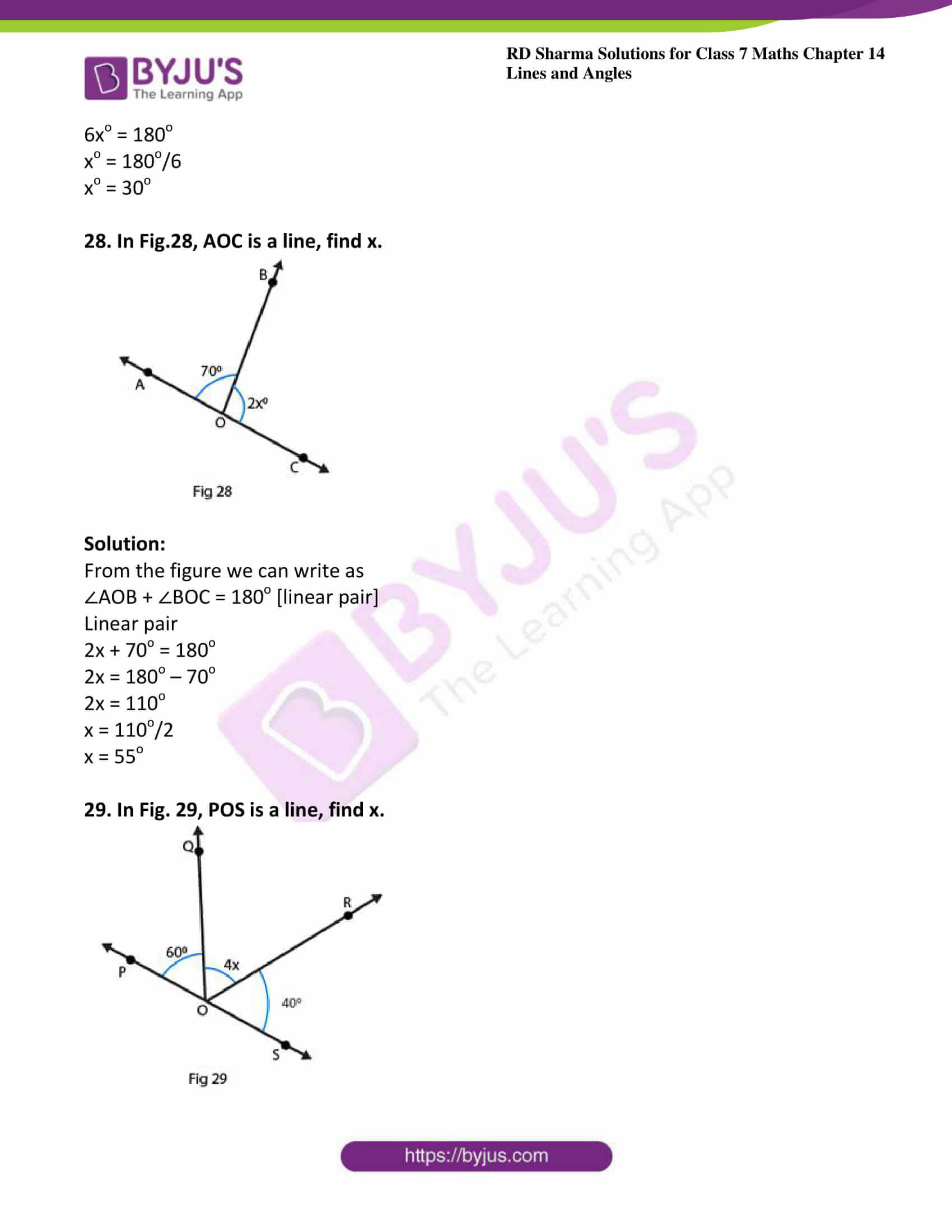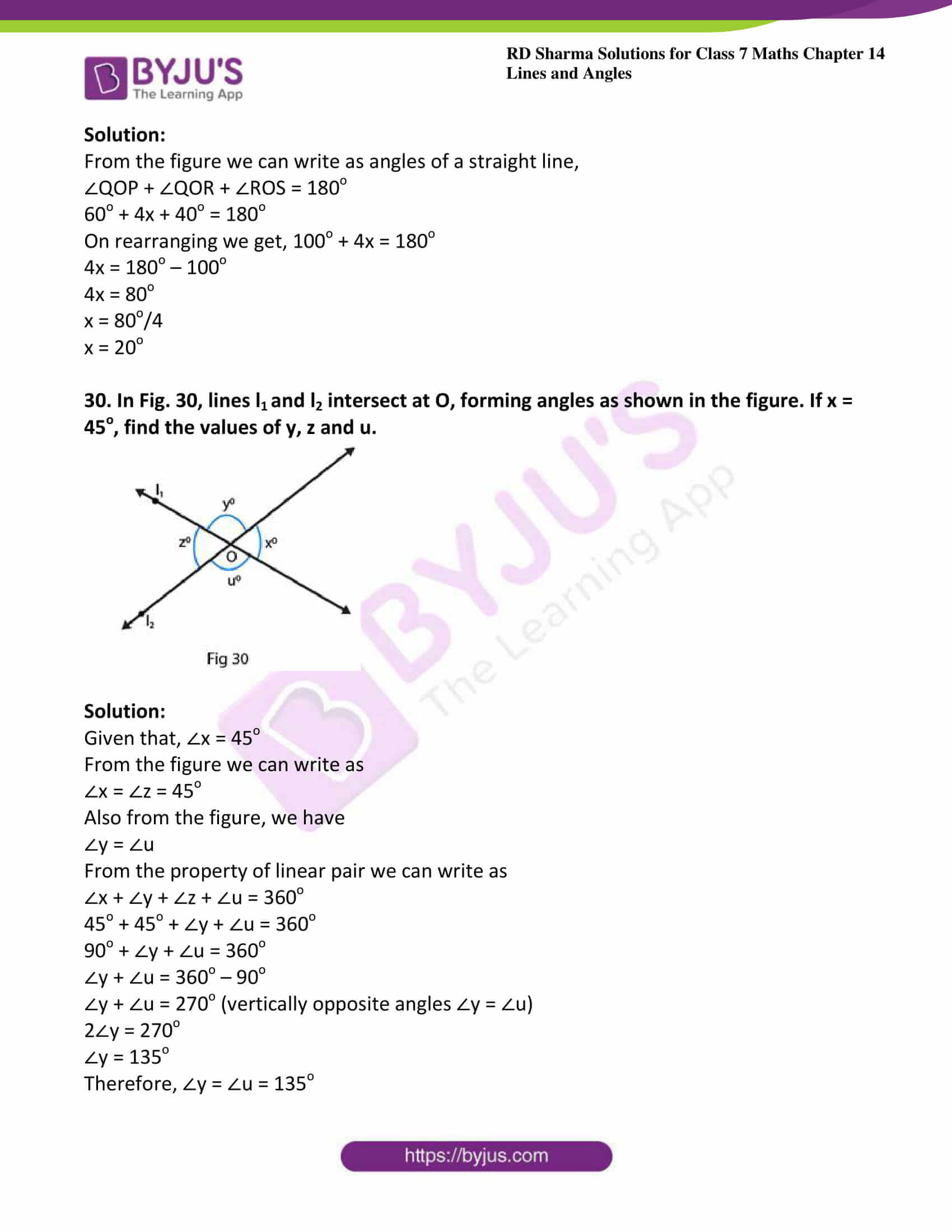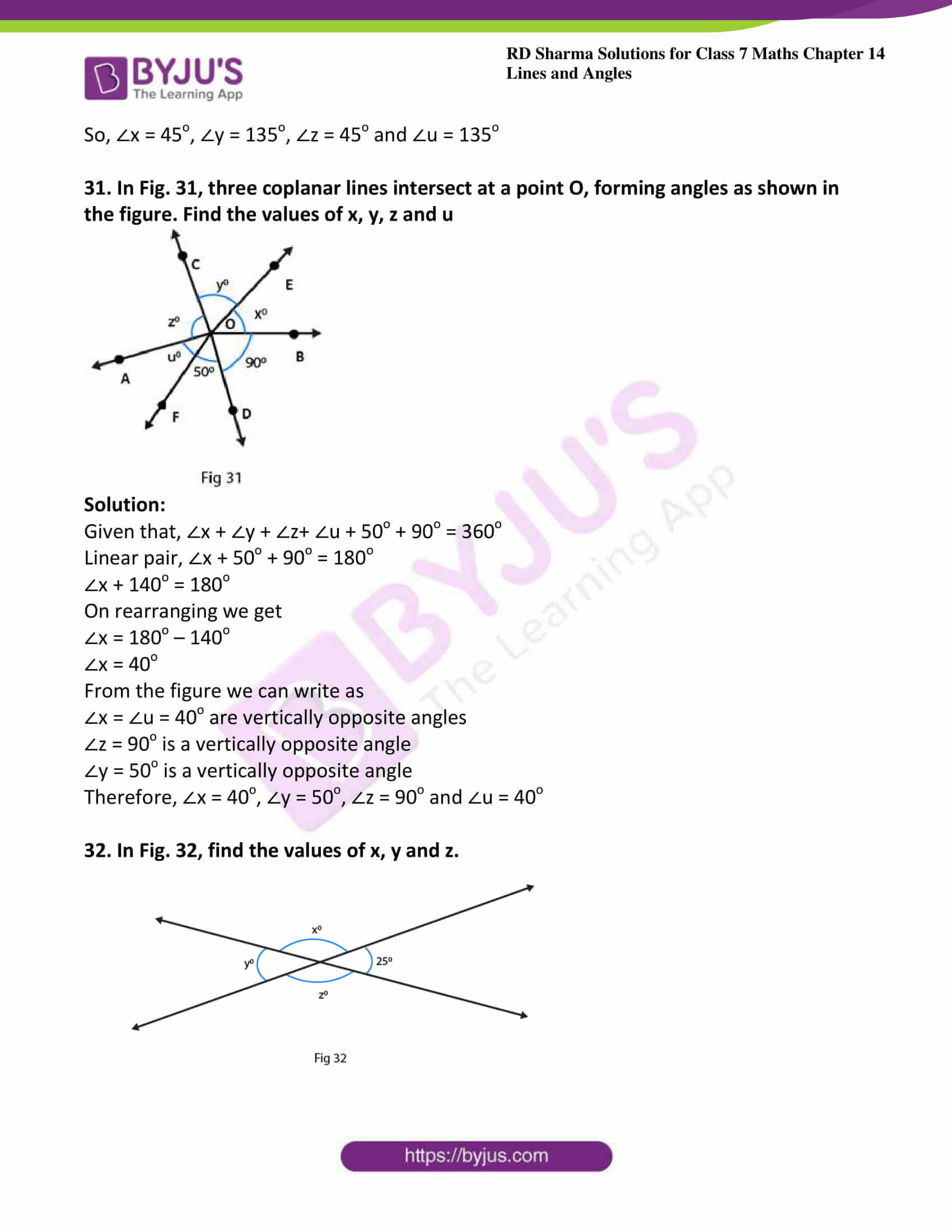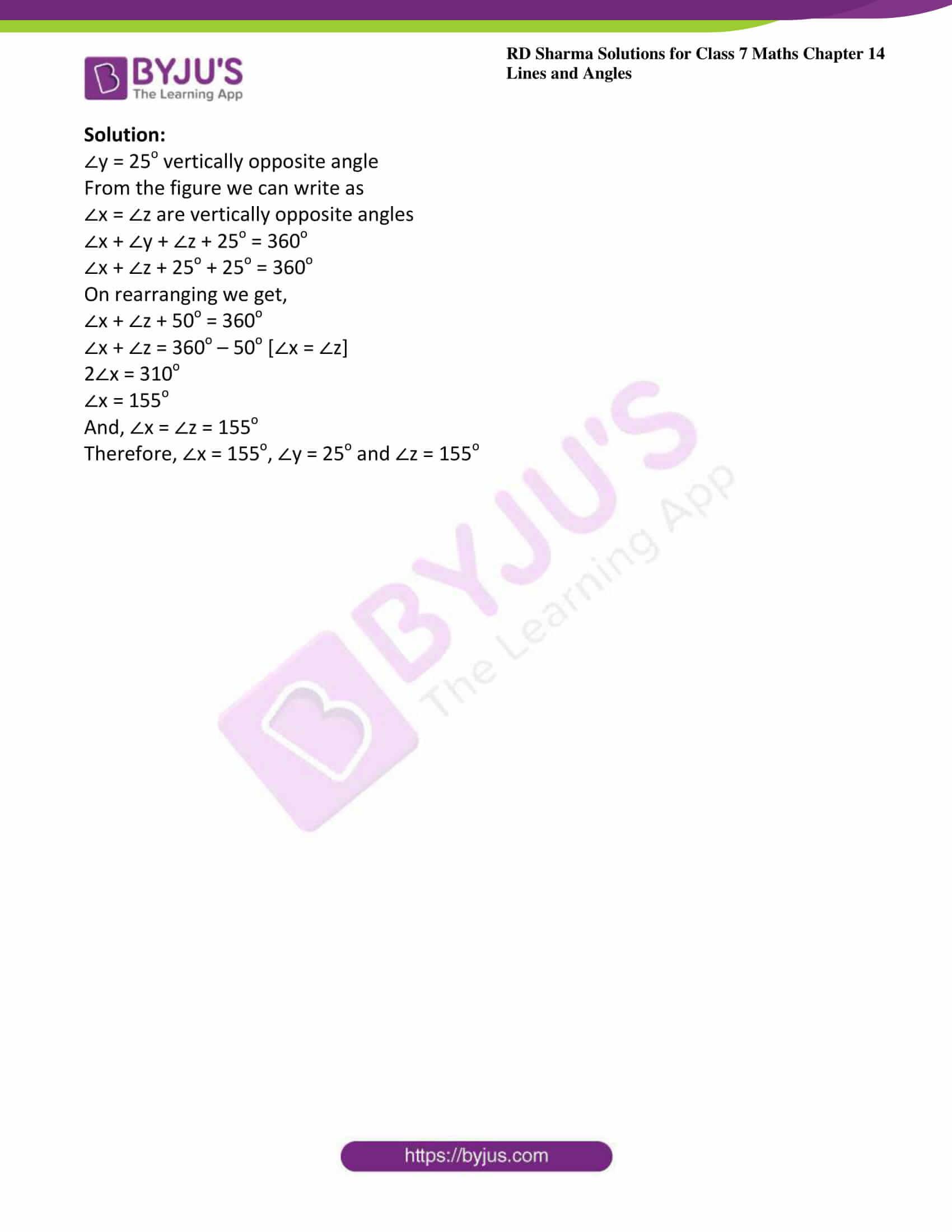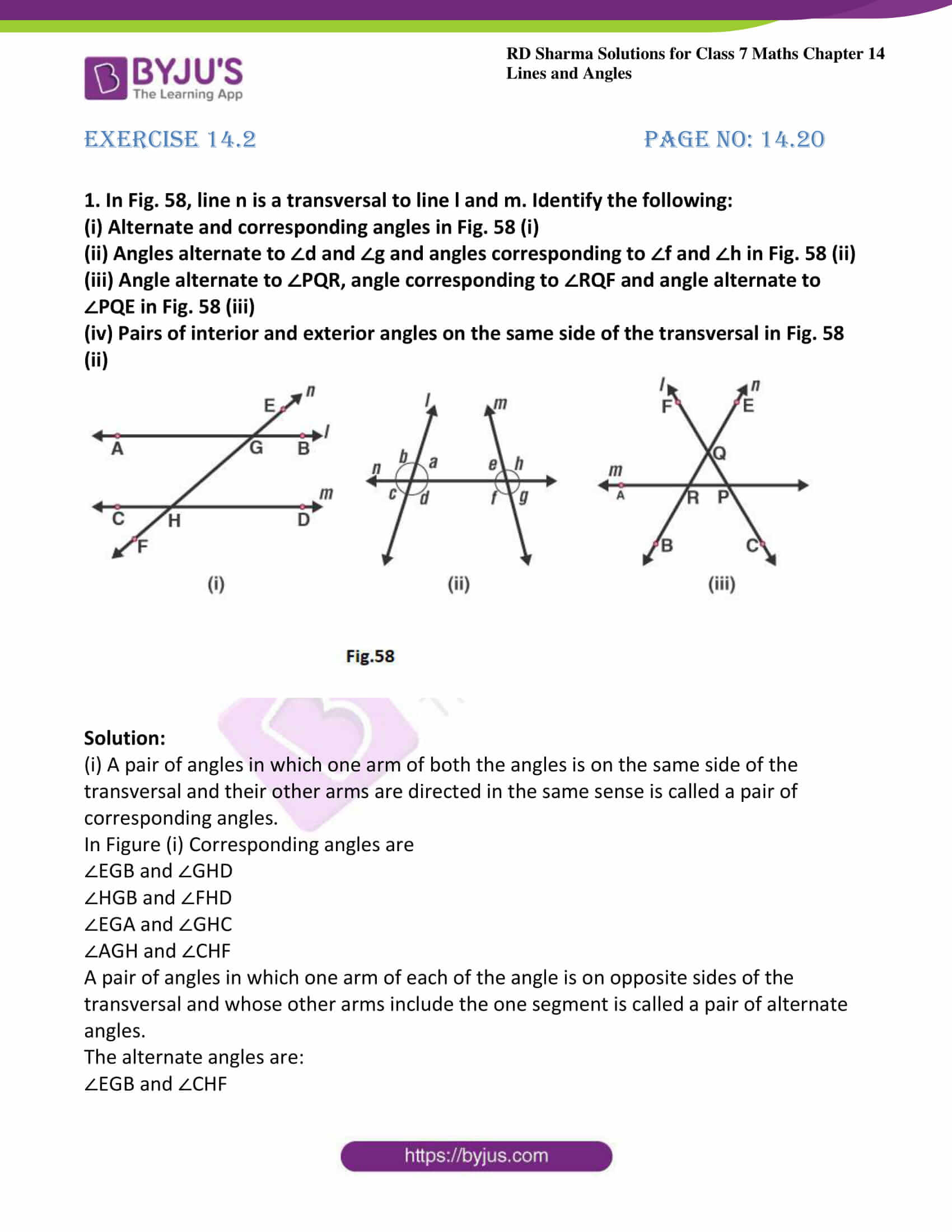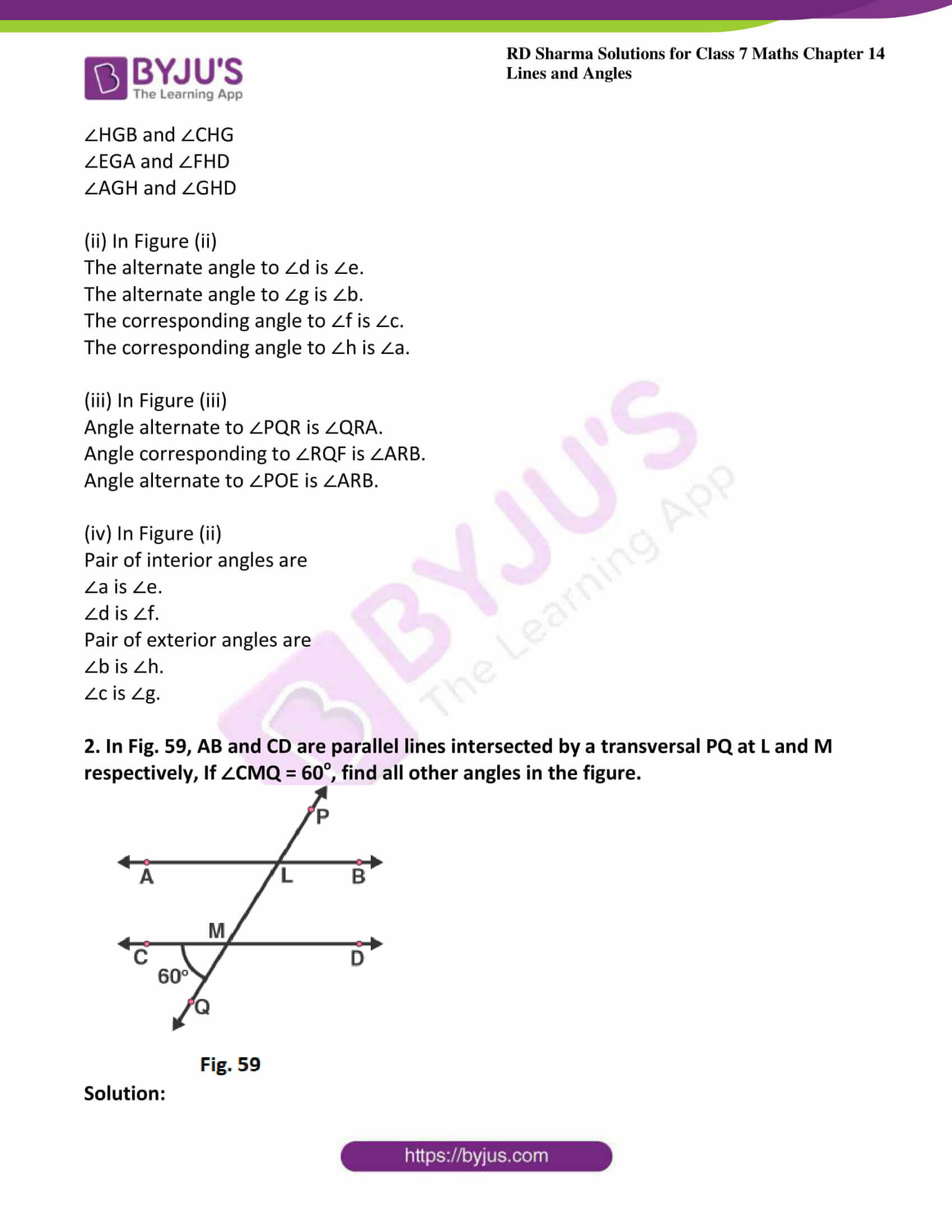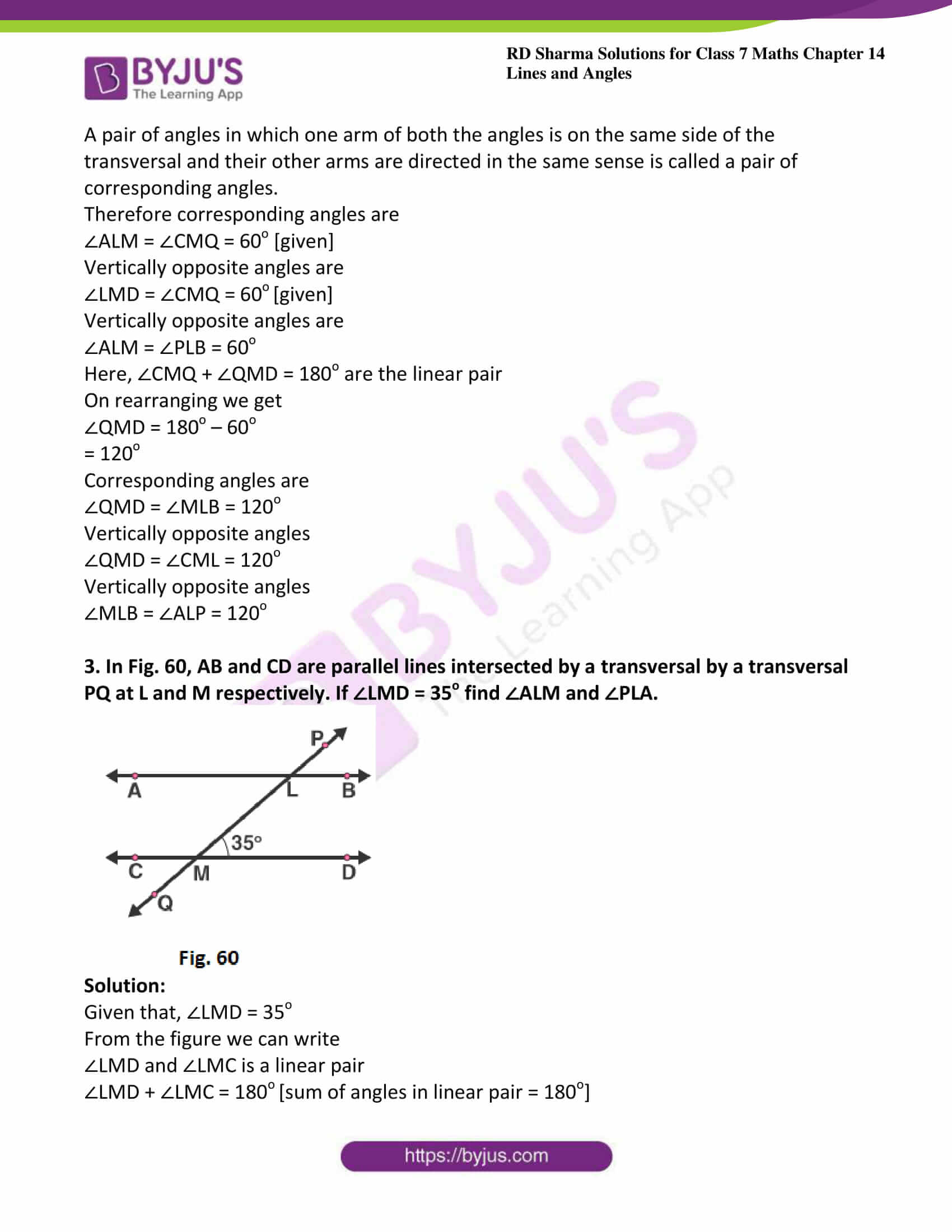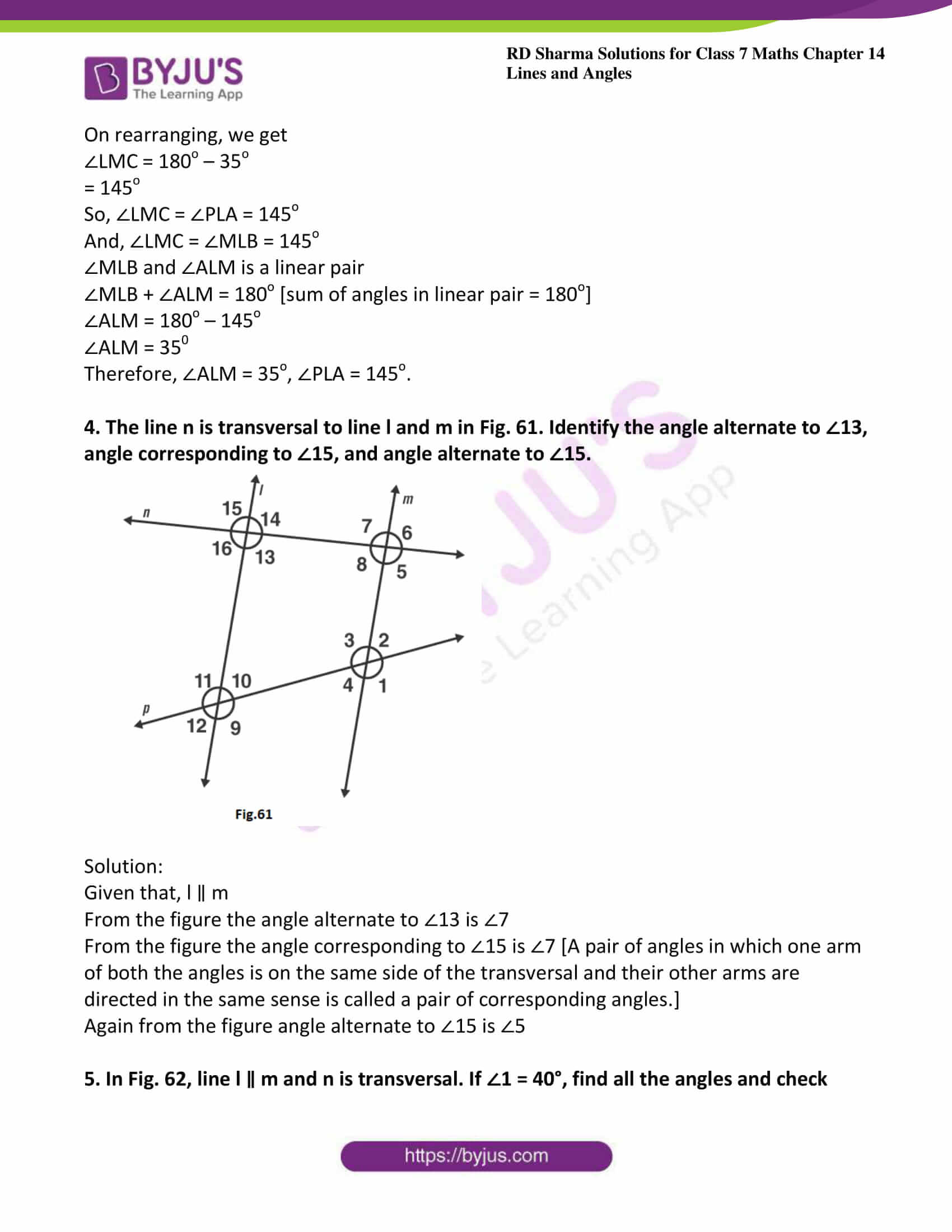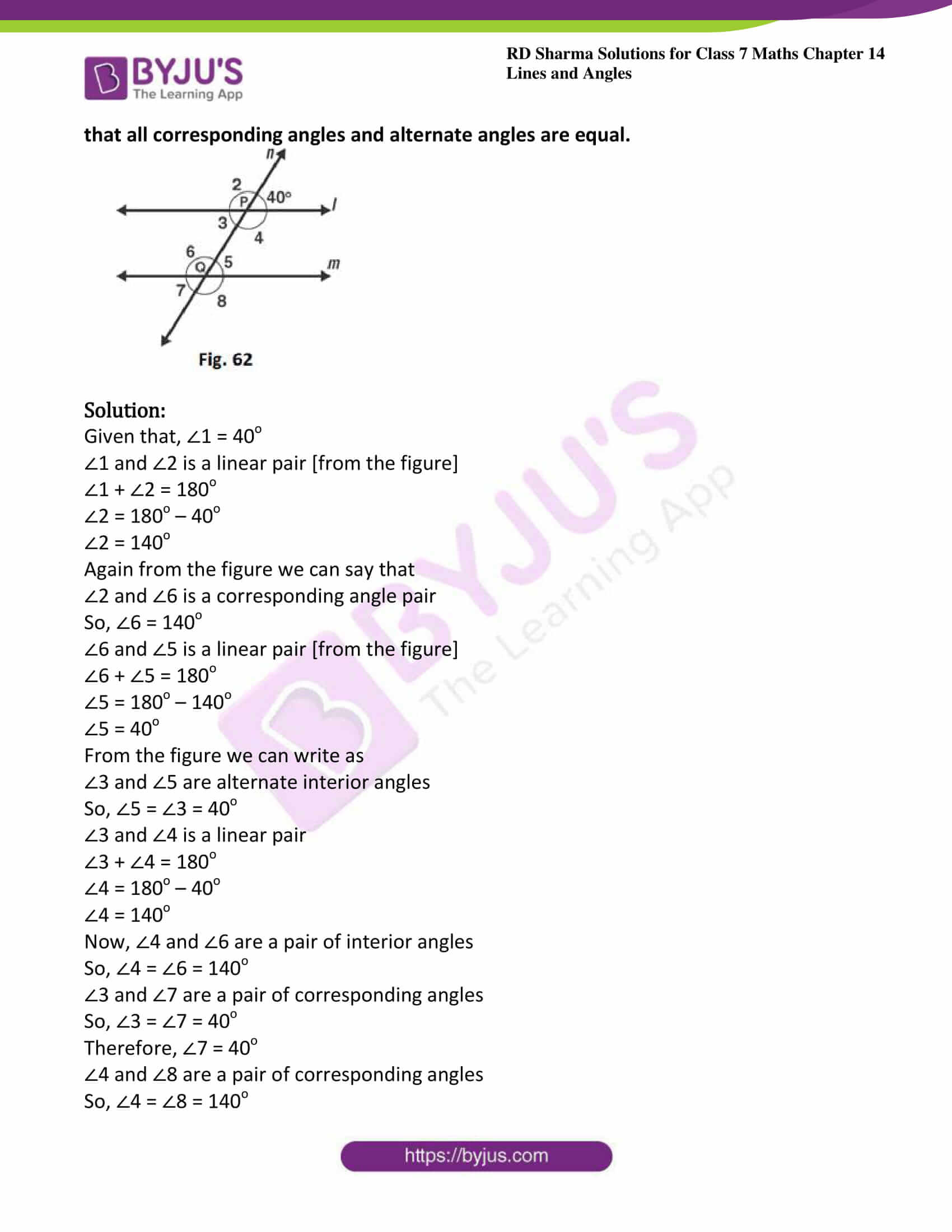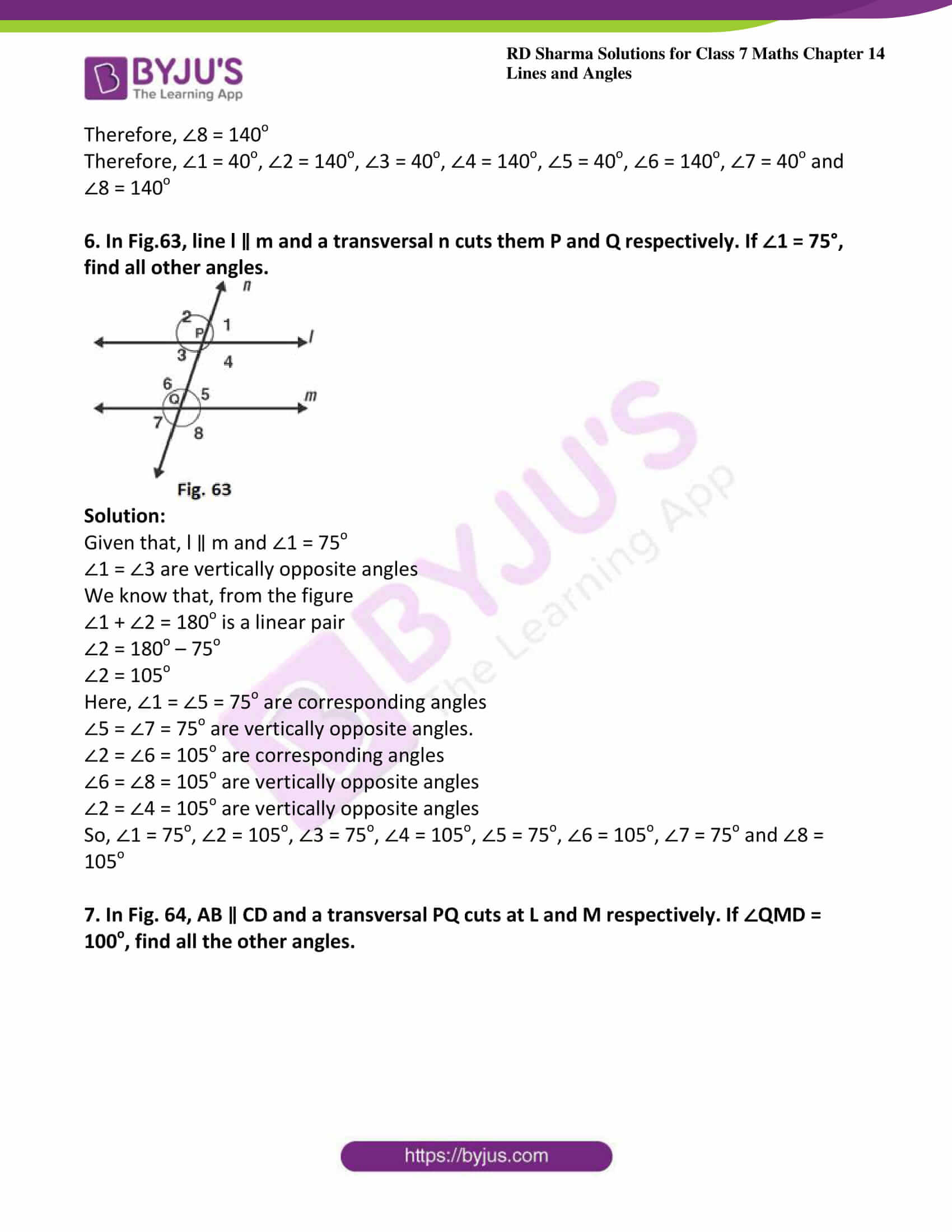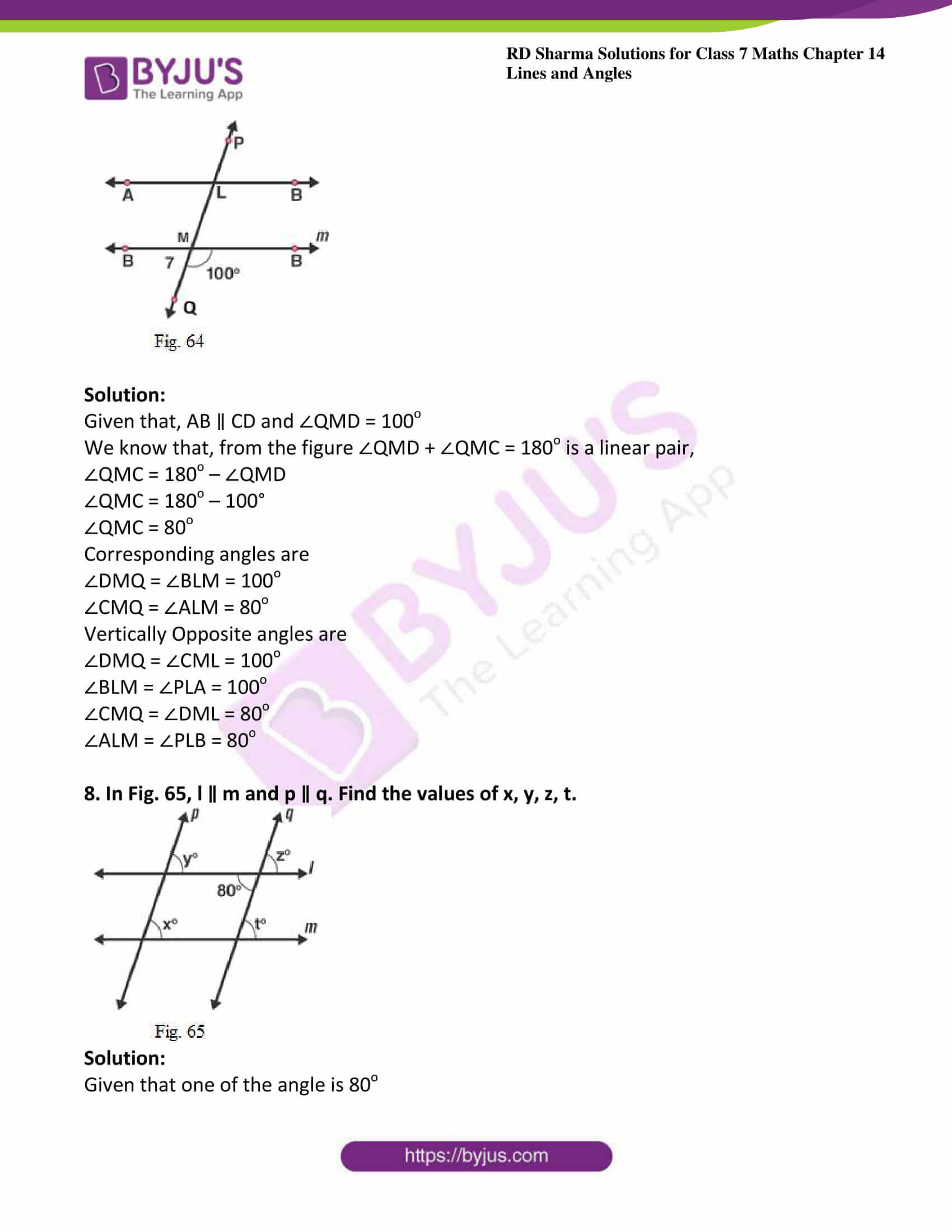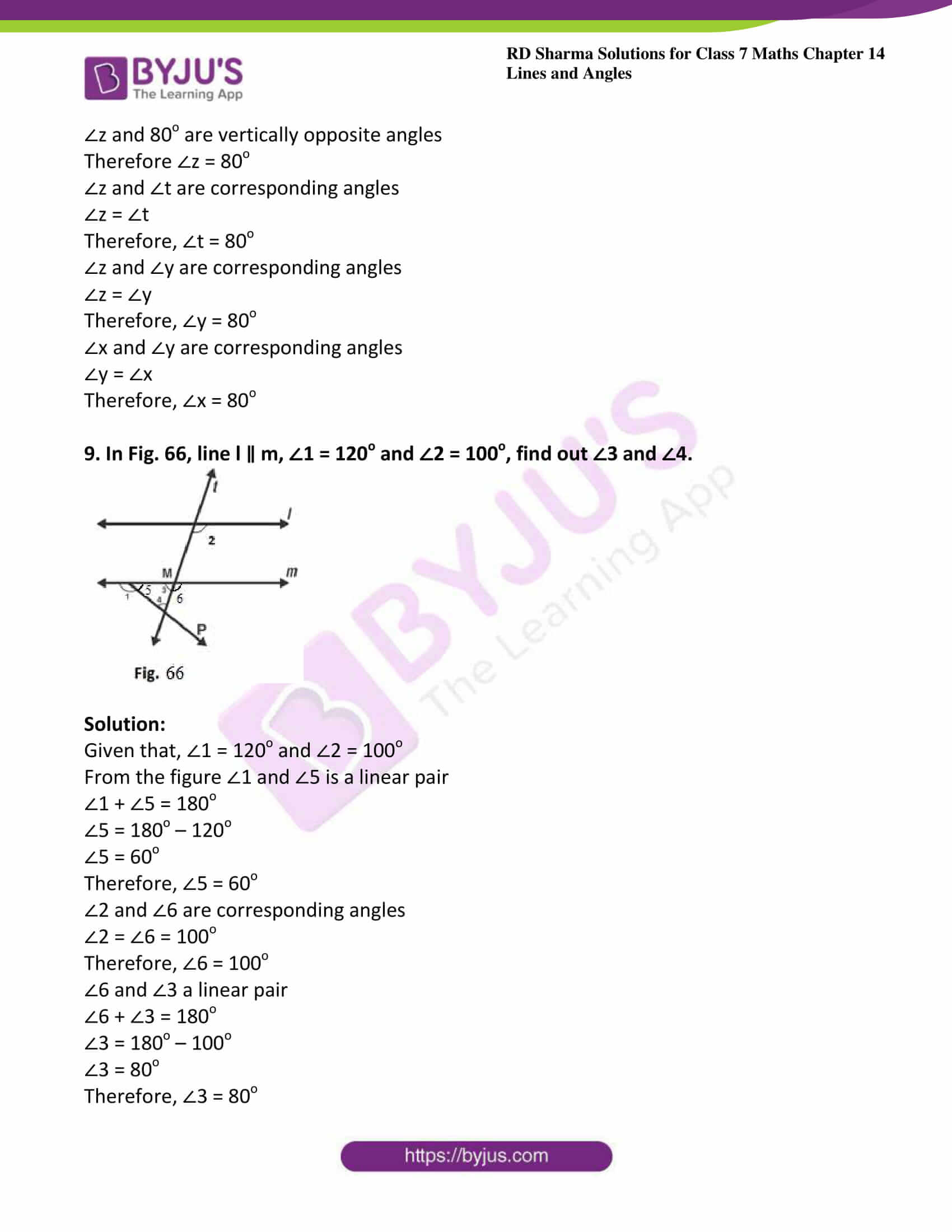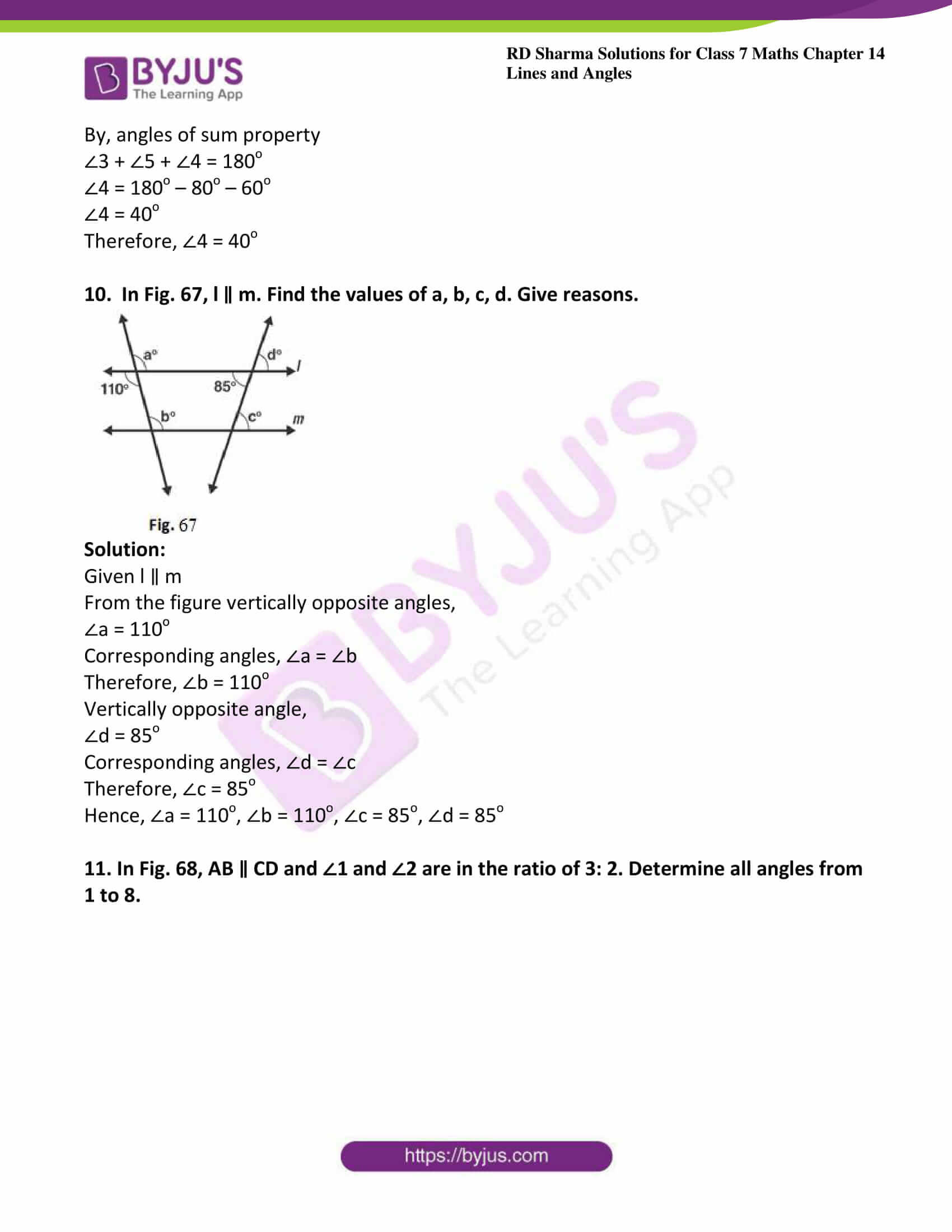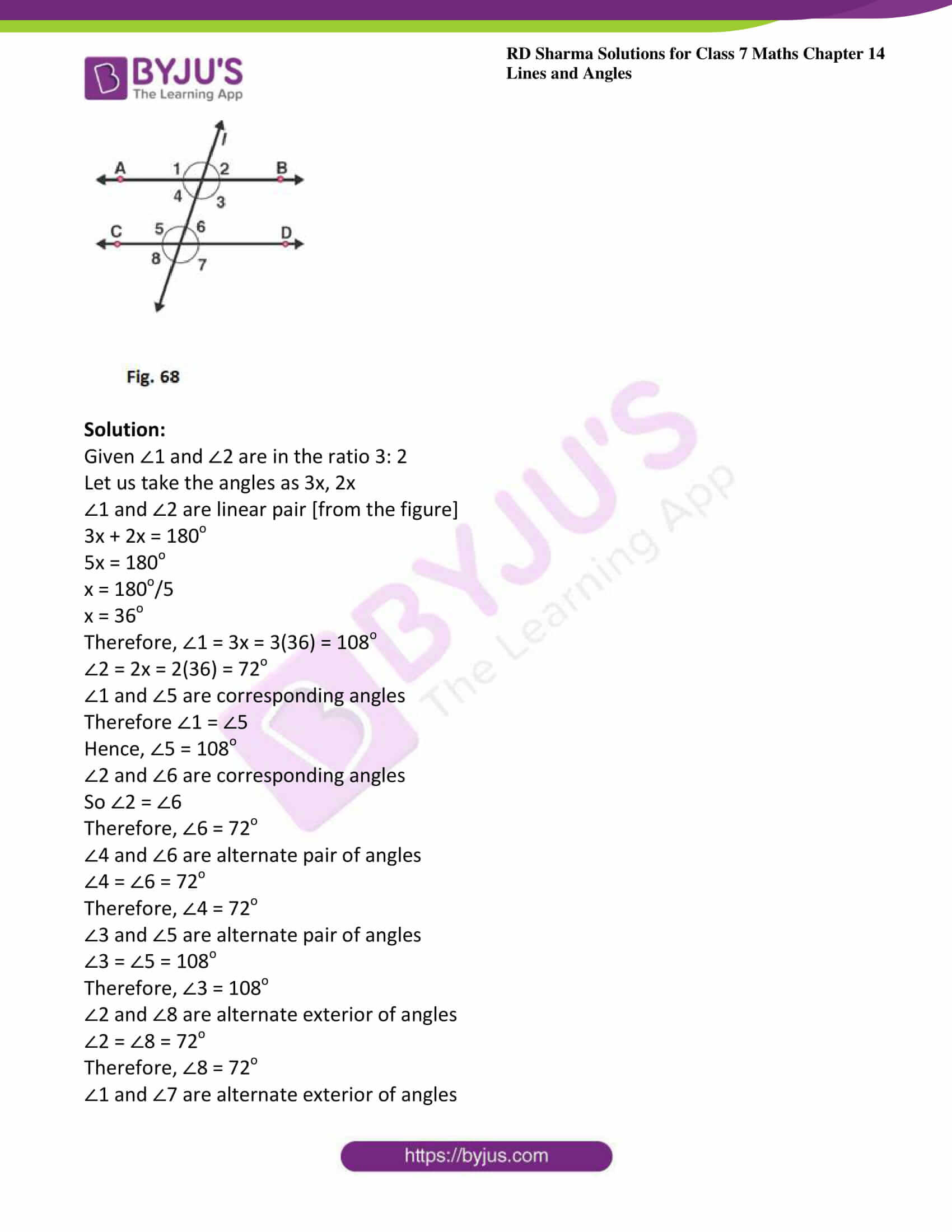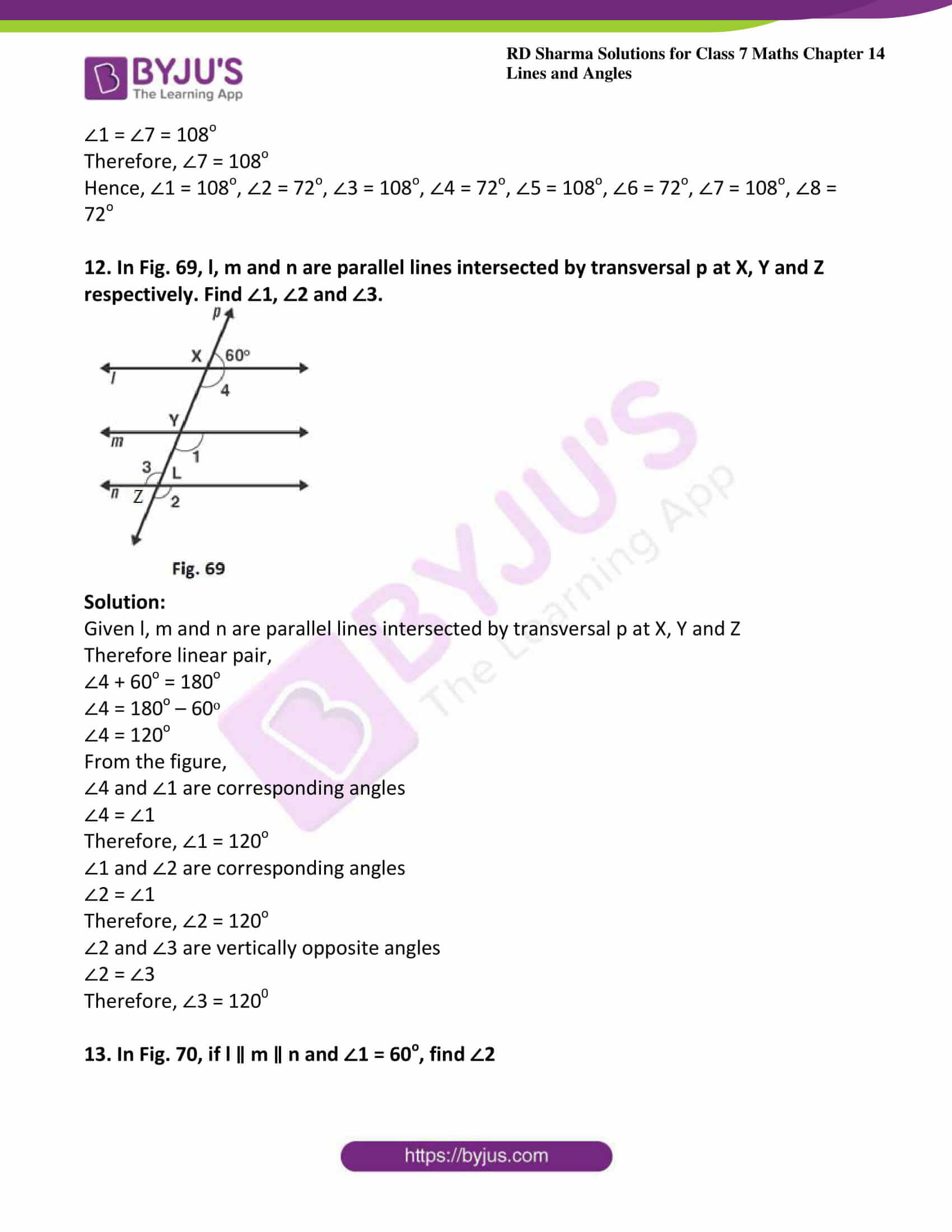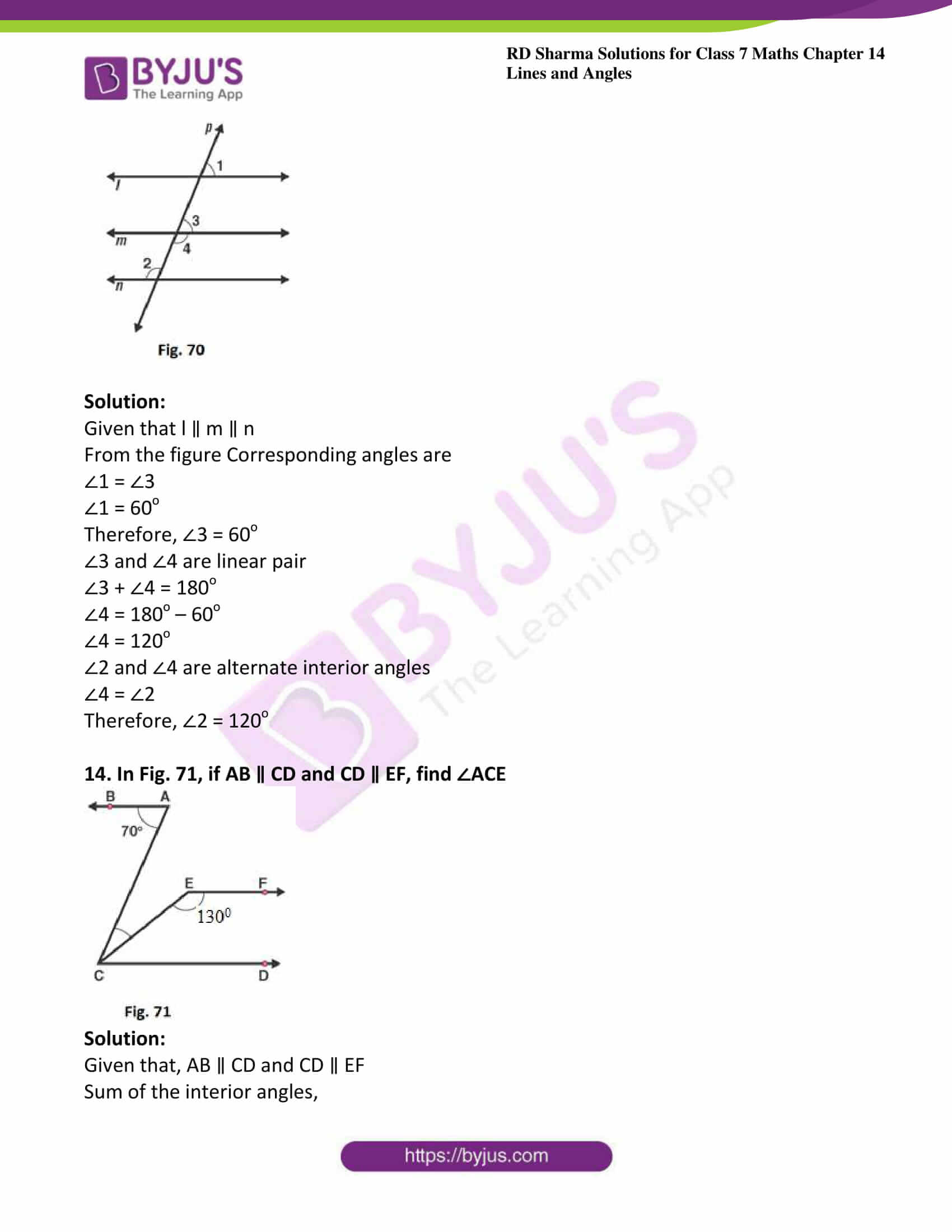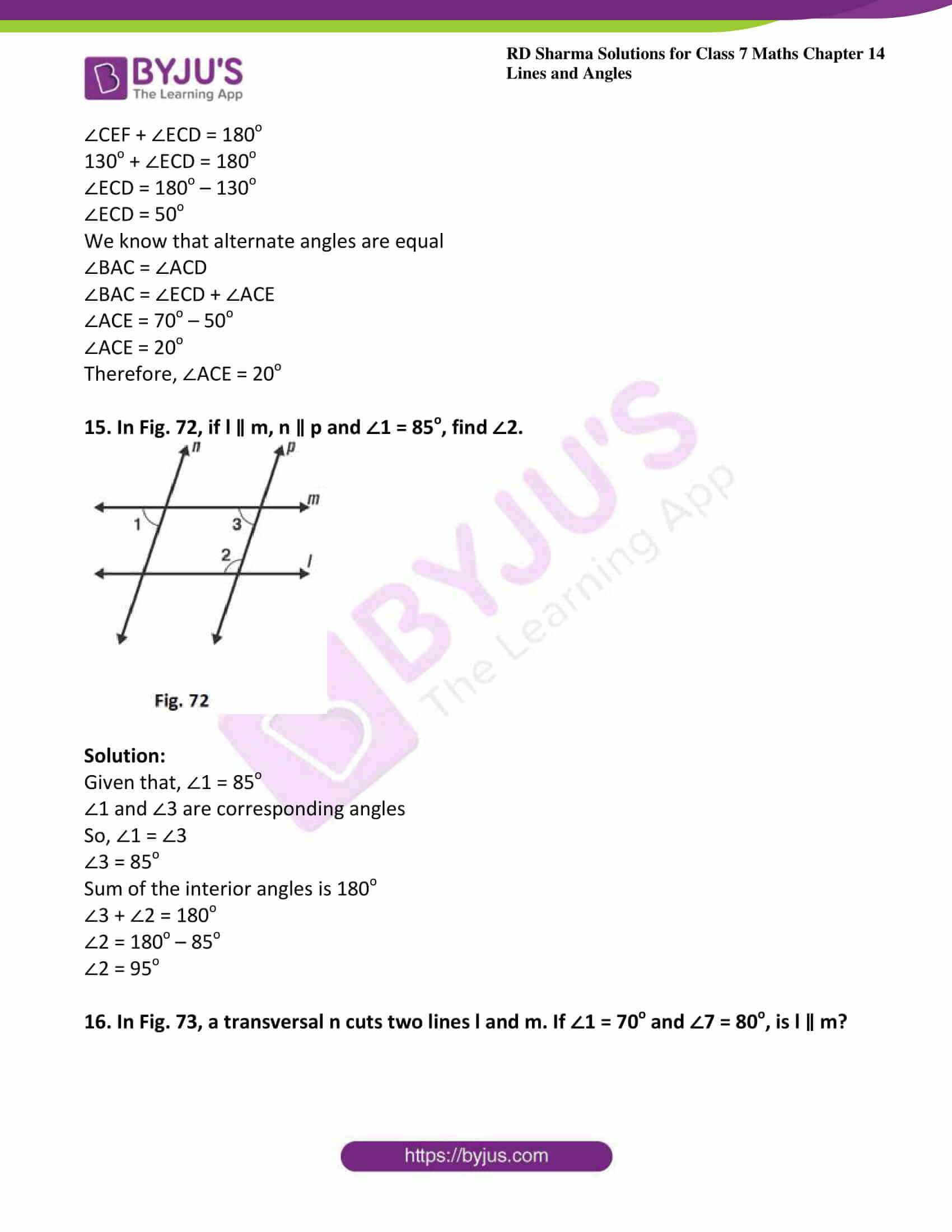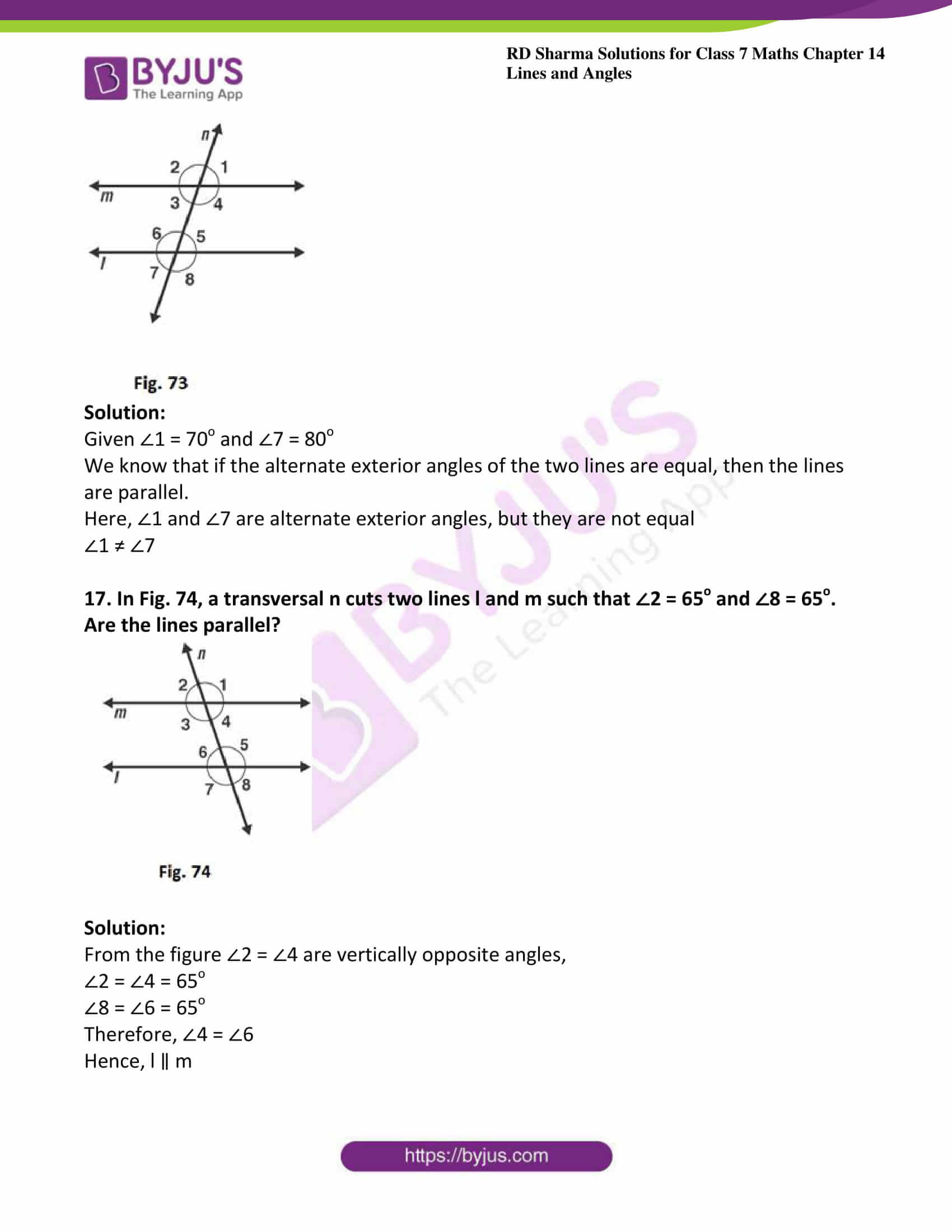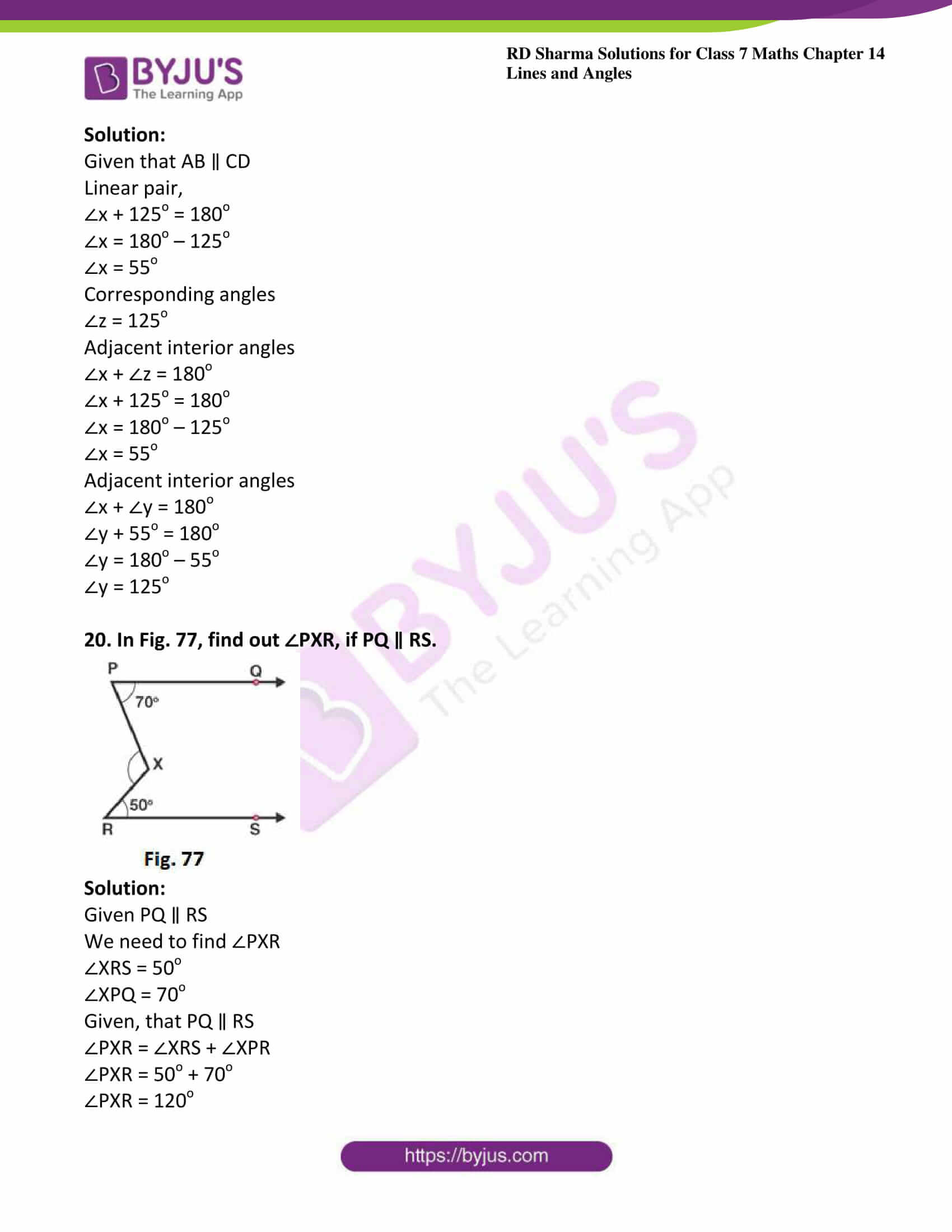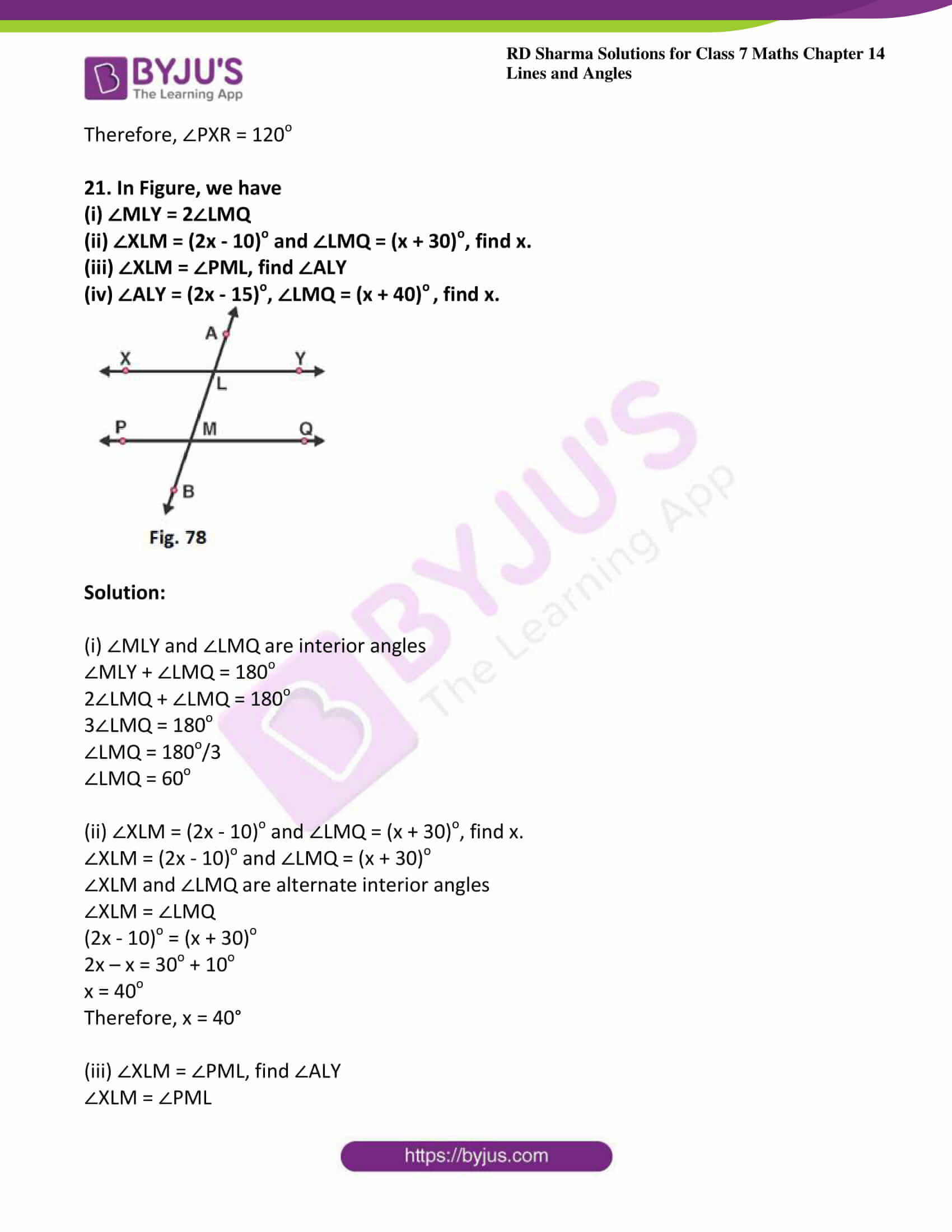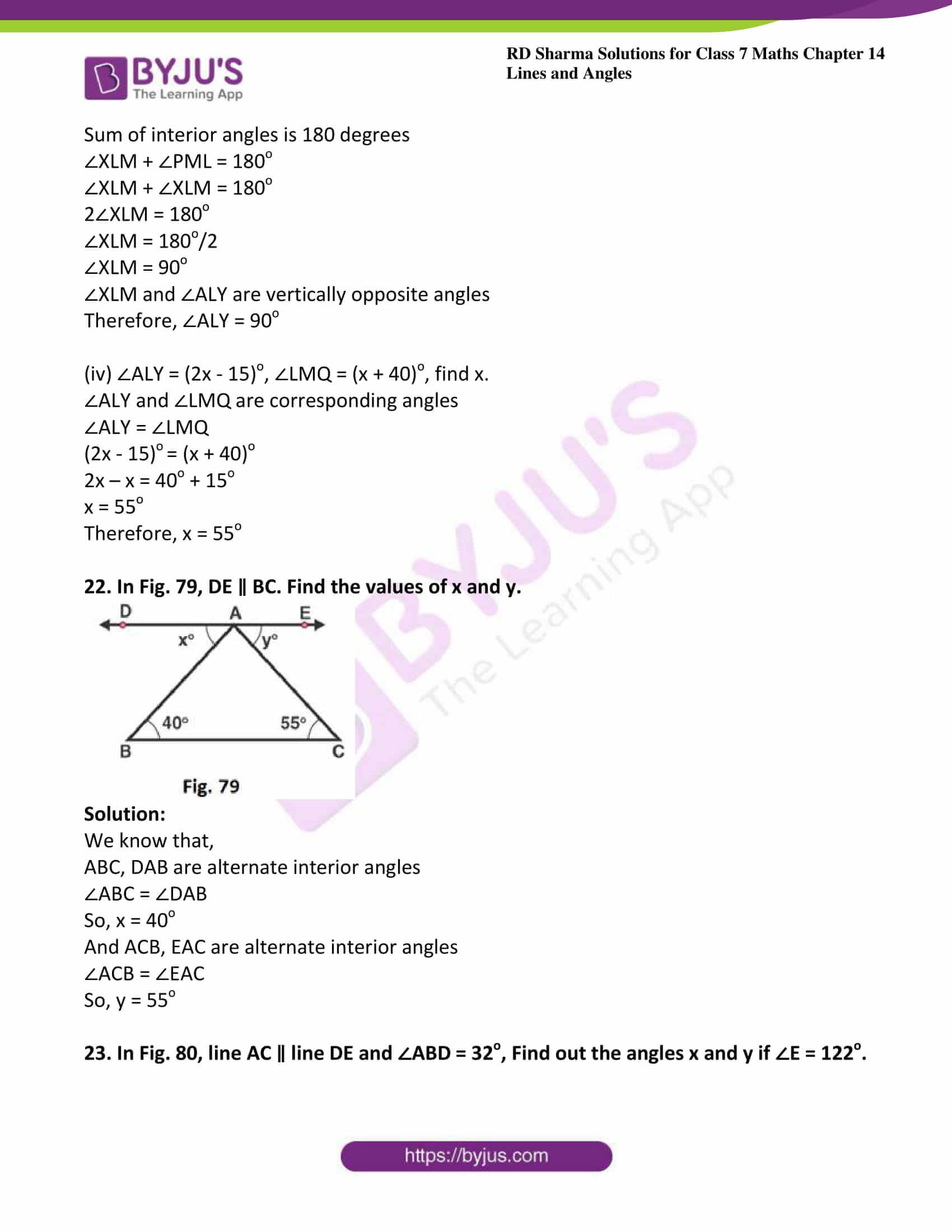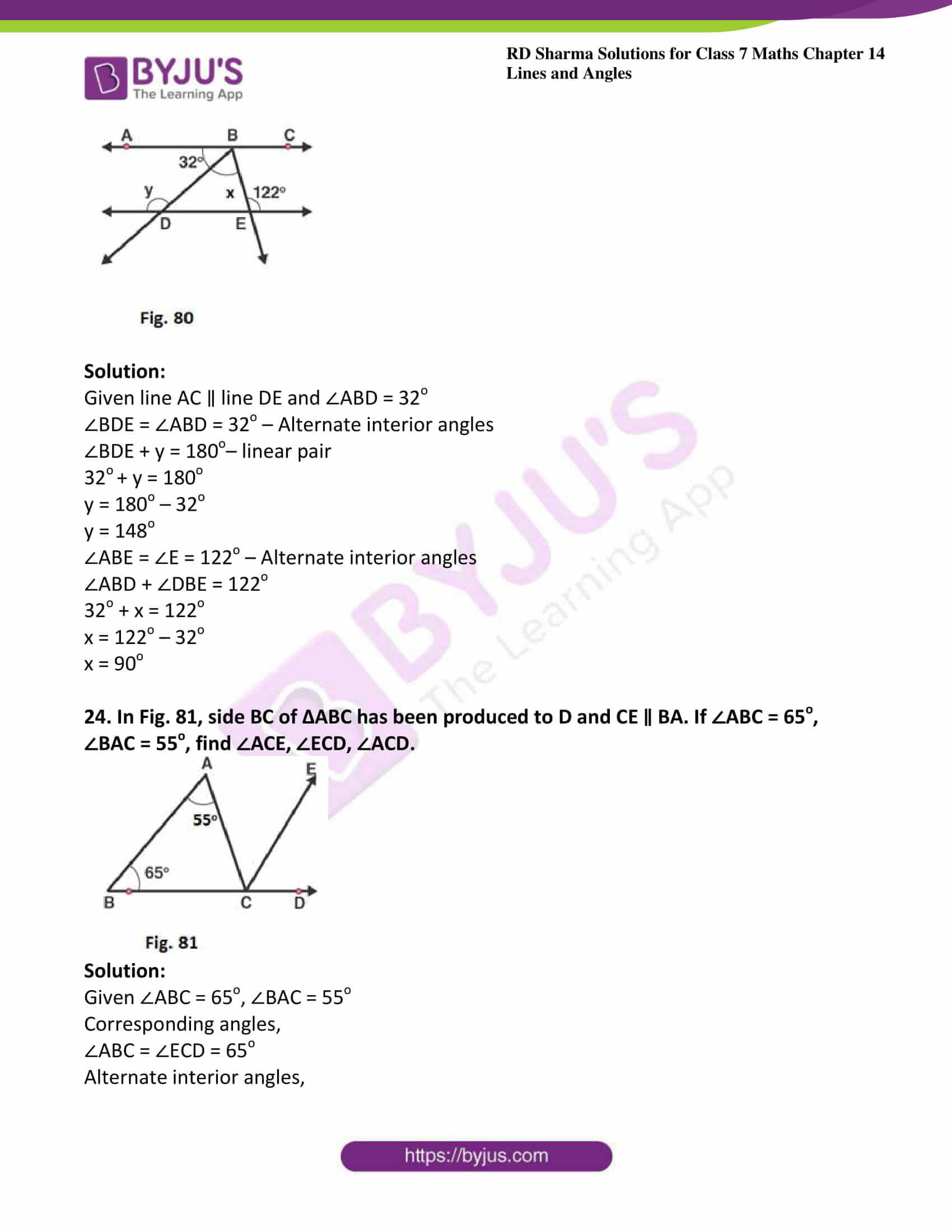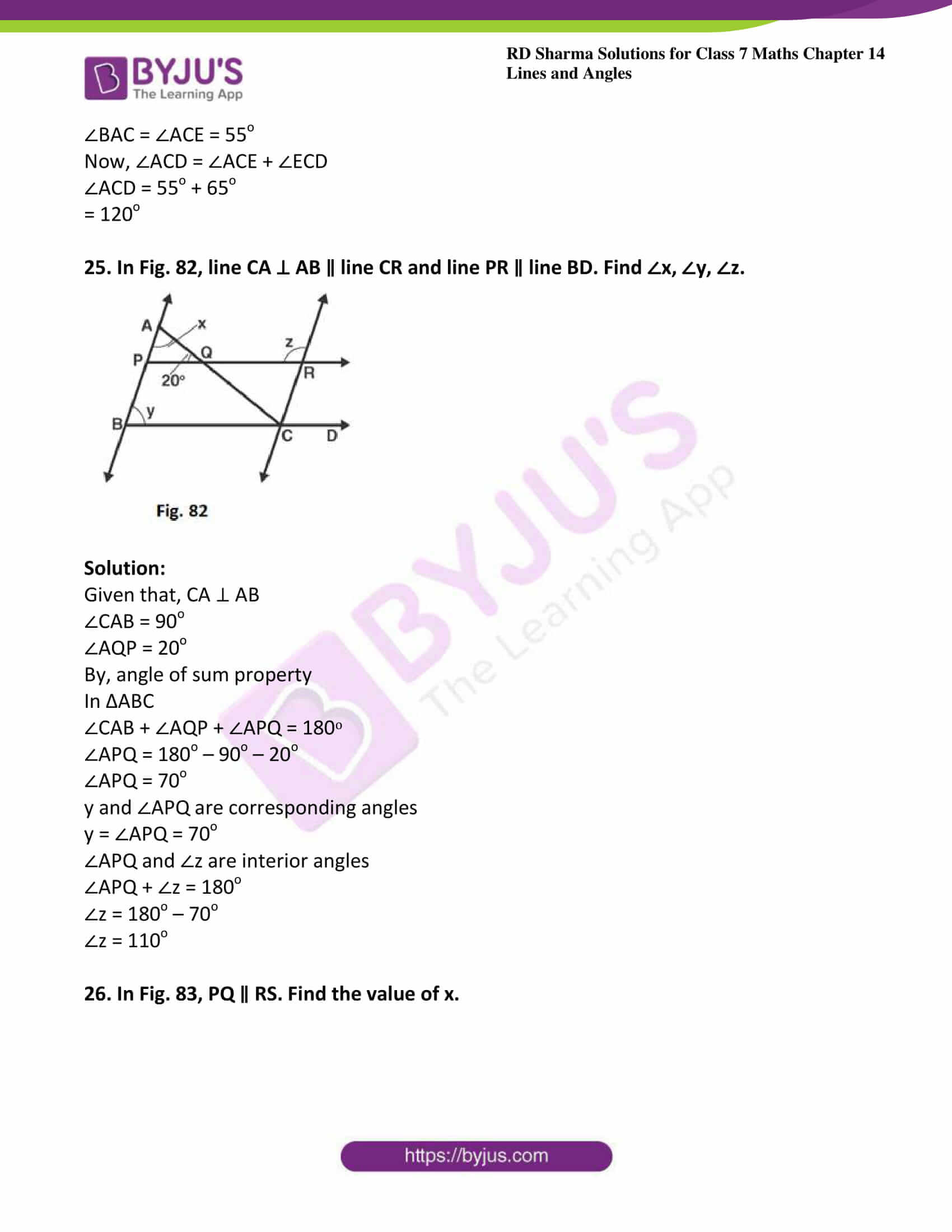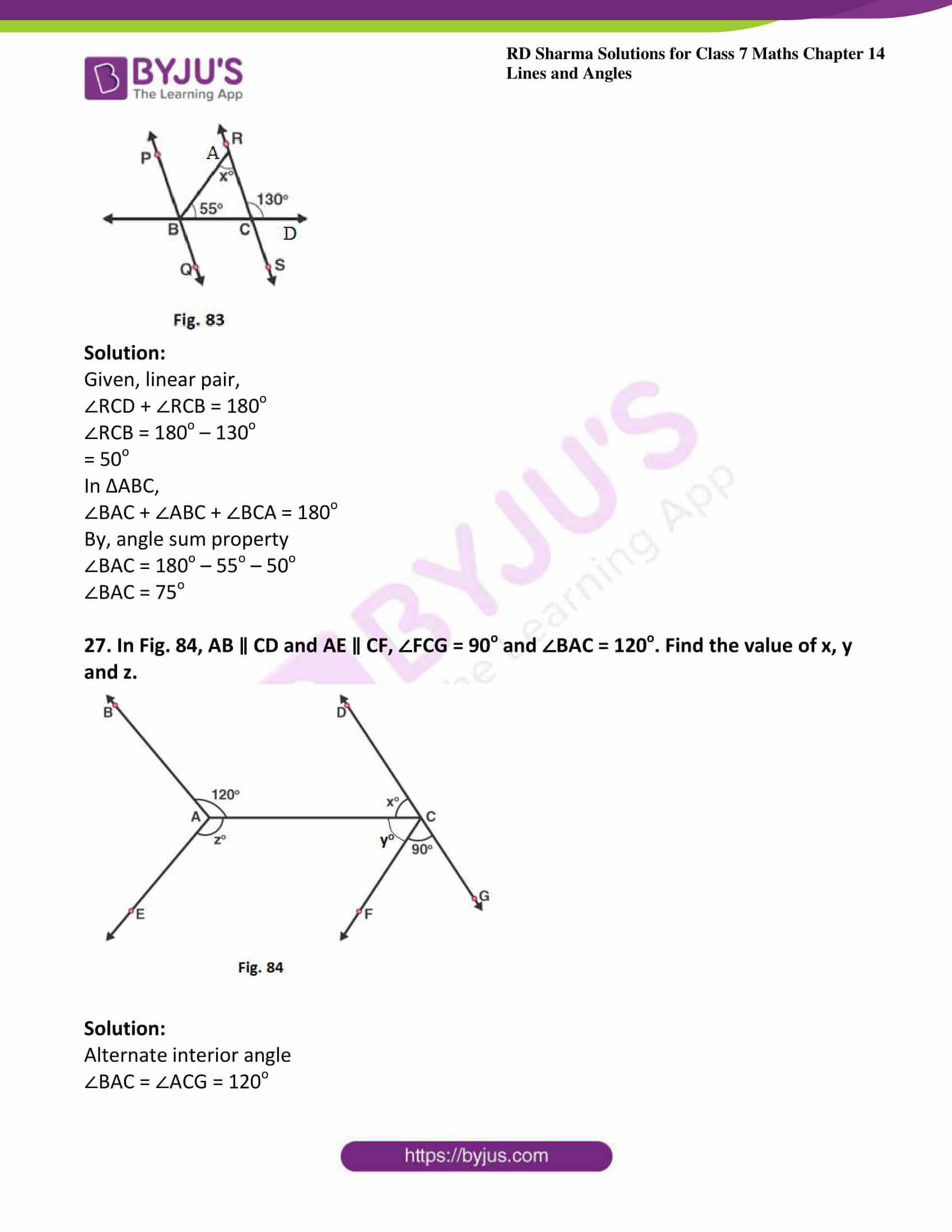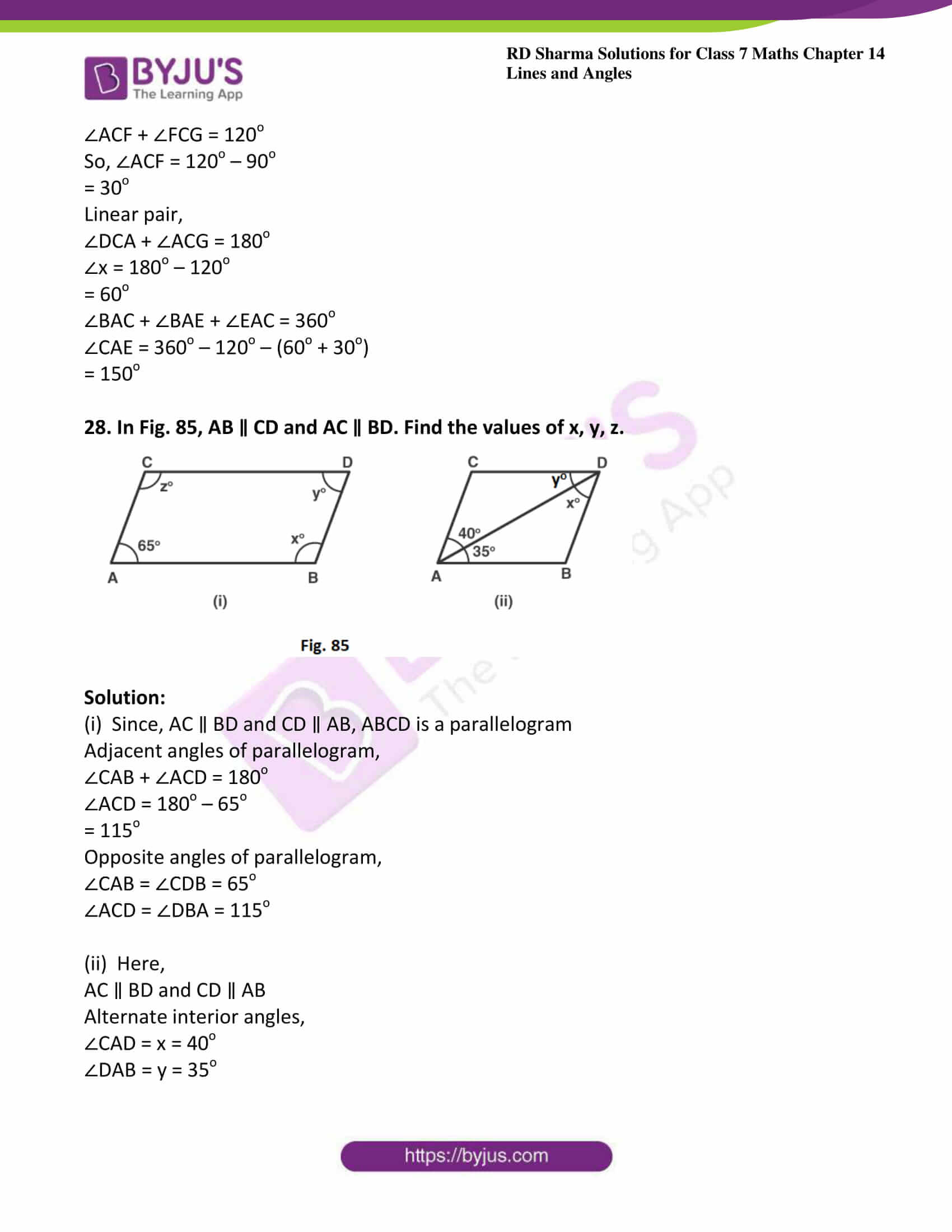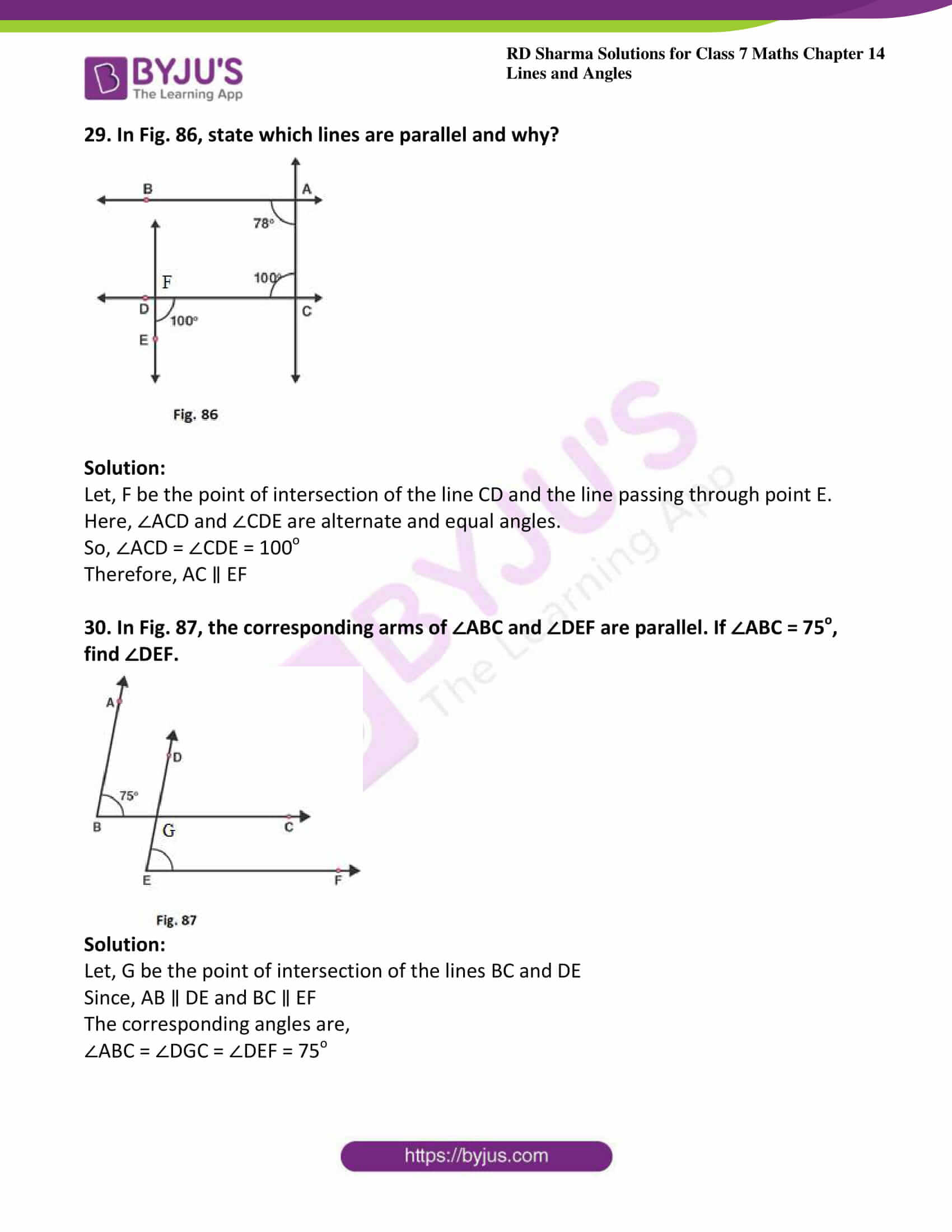### Access answers to Maths RD Sharma Solutions For Class 7 Chapter 14 – Lines And Angles

Exercise 14.1 Page No: 14.6

1. Write down each pair of adjacent angles shown in fig. 13.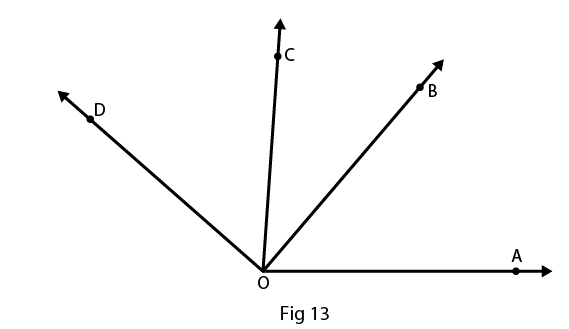Solution:

The angles that have common vertex and a common arm are known as adjacent angles

Therefore the adjacent angles in given figure are:

∠DOC and ∠BOC

∠COB and ∠BOA

2. In Fig. 14, name all the pairs of adjacent angles.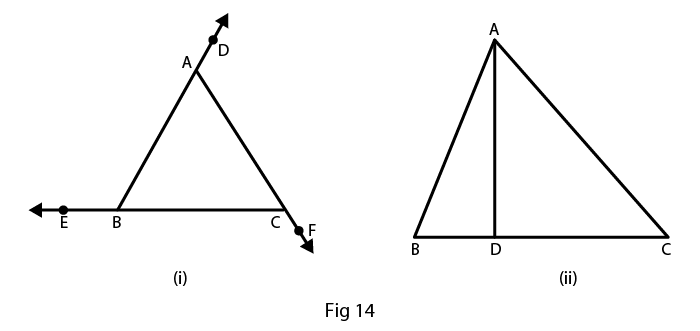Solution:

The angles that have common vertex and a common arm are known as adjacent angles.

In fig (i), the adjacent angles are

∠EBA and ∠ABC

∠ACB and ∠BCF

In fig (ii), the adjacent angles are

∠BDA and ∠CDA

3. In fig. 15, write down

(i) Each linear pair

(ii) Each pair of vertically opposite angles.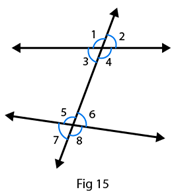Solution:

(i) The two adjacent angles are said to form a linear pair of angles if their non – common arms are two opposite rays.

∠1 and ∠3

∠1 and ∠2

∠4 and ∠3

∠4 and ∠2

∠5 and ∠6

∠5 and ∠7

∠6 and ∠8

∠7 and ∠8

(ii) The two angles formed by two intersecting lines and have no common arms are called vertically opposite angles.

∠1 and ∠4

∠2 and ∠3

∠5 and ∠8

∠6 and ∠7

4. Are the angles 1 and 2 given in Fig. 16 adjacent angles?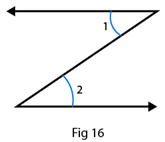Solution:

No, because they don’t have common vertex.

5. Find the complement of each of the following angles:

(i) 35o

(ii) 72o

(iii) 45o

(iv) 85o

Solution:

(i) The two angles are said to be complementary angles if the sum of those angles is 90o

Complementary angle for given angle is

90o – 35o = 55o

(ii) The two angles are said to be complementary angles if the sum of those angles is 90o

Complementary angle for given angle is

900 – 72o = 18o

(iii) The two angles are said to be complementary angles if the sum of those angles is 90o

Complementary angle for given angle is

90o – 45o = 45o

(iv) The two angles are said to be complementary angles if the sum of those angles is 90o

Complementary angle for given angle is

90o – 85o = 5o

6. Find the supplement of each of the following angles:

(i) 70o

(ii) 120o

(iii) 135o

(iv) 90o

Solution:

(i) The two angles are said to be supplementary angles if the sum of those angles is 180o

Therefore supplementary angle for the given angle is

180o – 70o = 110o

(ii) The two angles are said to be supplementary angles if the sum of those angles is 180o

Therefore supplementary angle for the given angle is

180o – 120o = 60o

(iii) The two angles are said to be supplementary angles if the sum of those angles is 180o

Therefore supplementary angle for the given angle is

180o – 135o = 45o

(iv) The two angles are said to be supplementary angles if the sum of those angles is 180o

Therefore supplementary angle for the given angle is

180o – 90o = 90o

7. Identify the complementary and supplementary pairs of angles from the following pairs:

(i) 25o, 65o

(ii) 120o, 60o

(iii) 63o, 27o

(iv) 100o, 80o

Solution:

(i) 25o + 65o = 90o so, this is a complementary pair of angle.

(ii) 120o + 60o = 180o so, this is a supplementary pair of angle.

(iii) 63o + 27o = 90o so, this is a complementary pair of angle.

(iv) 100o + 80o = 180o so, this is a supplementary pair of angle.

8. Can two obtuse angles be supplementary, if both of them be

(i) Obtuse?

(ii) Right?

(iii) Acute?

Solution:

(i) No, two obtuse angles cannot be supplementary

Because, the sum of two angles is greater than 90o so their sum will be greater than 180o

(ii) Yes, two right angles can be supplementary

Because, 90o + 90o = 180o

(iii) No, two acute angle cannot be supplementary

Because, the sum of two angles is less than 90o so their sum will also be less than 90o

9. Name the four pairs of supplementary angles shown in Fig.17.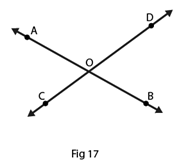Solution:

The two angles are said to be supplementary angles if the sum of those angles is 180o

The supplementary angles are

∠AOC and ∠COB

∠BOC and ∠DOB

∠BOD and ∠DOA

∠AOC and ∠DOA

10. In Fig. 18, A, B, C are collinear points and ∠DBA = ∠EBA.

(i) Name two linear pairs.

(ii) Name two pairs of supplementary angles.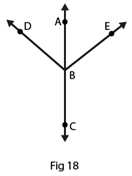Solution:

(i) Two adjacent angles are said to be form a linear pair of angles, if their non-common arms are two opposite rays.

Therefore linear pairs are

∠ABD and ∠DBC

∠ABE and ∠EBC

(ii) We know that every linear pair forms supplementary angles, these angles are

∠ABD and ∠DBC

∠ABE and ∠EBC

11. If two supplementary angles have equal measure, what is the measure of each angle?

Solution:

Let p and q be the two supplementary angles that are equal

The two angles are said to be supplementary angles if the sum of those angles is 180o

∠p = ∠q

So,

∠p + ∠q = 180o

∠p + ∠p = 180o

2∠p = 180o

∠p = 180o/2

∠p = 90o

Therefore, ∠p = ∠q = 90o

12. If the complement of an angle is 28o, then find the supplement of the angle.

Solution:

Given complement of an angle is 28o

Here, let x be the complement of the given angle 28o

Therefore, ∠x + 28o = 90o

∠x = 90o – 28o

= 62o

So, the supplement of the angle = 180o – 62o

= 118o

13. In Fig. 19, name each linear pair and each pair of vertically opposite angles: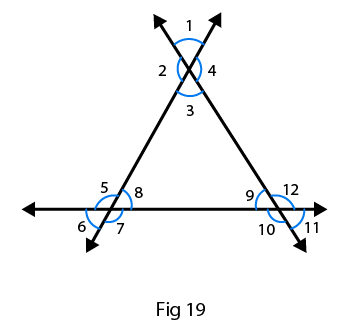Solution:

Two adjacent angles are said to be linear pair of angles, if their non-common arms are two opposite rays.

Therefore linear pairs are listed below:

∠1 and ∠2

∠2 and ∠3

∠3 and ∠4

∠1 and ∠4

∠5 and ∠6

∠6 and ∠7

∠7 and ∠8

∠8 and ∠5

∠9 and ∠10

∠10 and ∠11

∠11 and ∠12

∠12 and ∠9

The two angles are said to be vertically opposite angles if the two intersecting lines have no common arms.

Therefore supplement of the angle are listed below:

∠1 and ∠3

∠4 and ∠2

∠5 and ∠7

∠6 and ∠8

∠9 and ∠11

∠10 and ∠12

14. In Fig. 20, OE is the bisector of ∠BOD. If ∠1 = 70o, find the magnitude of ∠2, ∠3 and ∠4.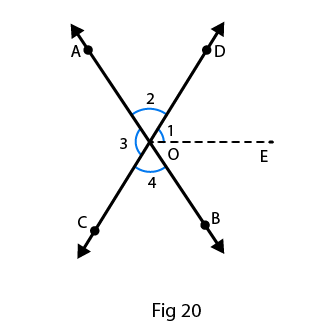Solution:

Given, ∠1 = 70o

∠3 = 2(∠1)

= 2(70o)

∠3 = 140o

∠3 = ∠4

As, OE is the angle bisector,

∠DOB = 2(∠1)

= 2(70o)

= 140o

∠DOB + ∠AOC + ∠COB +∠AOD = 360o [sum of the angle of circle = 360o]

140o + 140o + 2(∠COB) = 360o

Since, ∠COB = ∠AOD

2(∠COB) = 360o – 280o

2(∠COB) = 80o

∠COB = 80o/2

∠COB = 40o

Therefore, ∠COB = ∠AOD = 40o

The angles are, ∠1 = 70o, ∠2 = 40o, ∠3 = 140o and ∠4 = 40o

15. One of the angles forming a linear pair is a right angle. What can you say about its other angle?

Solution:

Given one of the angle of a linear pair is the right angle that is 90o

We know that linear pair angle is 180o

Therefore, the other angle is

180o – 90o = 90o

16. One of the angles forming a linear pair is an obtuse angle. What kind of angle is the other?

Solution:

Given one of the angles of a linear pair is obtuse, then the other angle should be acute, because only then their sum will be 180o.

17. One of the angles forming a linear pair is an acute angle. What kind of angle is the other?

Solution:

Given one of the Angles of a linear pair is acute, then the other angle should be obtuse, only then their sum will be 180o.

18. Can two acute angles form a linear pair?

Solution:

No, two acute angles cannot form a linear pair because their sum is always less than 180o.

19. If the supplement of an angle is 65o, then find its complement.

Solution:

Let x be the required angle

So, x + 65o = 180o

x = 180o – 65o

x = 115o

The two angles are said to be complementary angles if the sum of those angles is 90o here it is more than 90o therefore the complement of the angle cannot be determined.

20. Find the value of x in each of the following figures.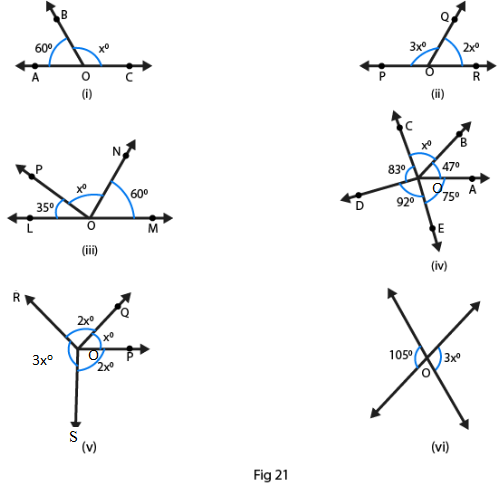Solution:

(i)  We know that ∠BOA + ∠BOC = 180o

[Linear pair: The two adjacent angles are said to form a linear pair of angles if their non–common arms are two opposite rays and sum of the angle is 180o]

60o + xo = 180o

xo = 180o – 60o

xo = 120o

(ii) We know that ∠POQ + ∠QOR = 180o

[Linear pair: The two adjacent angles are said to form a linear pair of angles if their non–common arms are two opposite rays and sum of the angle is 180o]

3xo + 2xo = 180o

5xo = 180o

xo = 180o/5

xo = 36o

(iii) We know that ∠LOP + ∠PON + ∠NOM = 180o

[Linear pair: The two adjacent angles are said to form a linear pair of angles if their non–common arms are two opposite rays and sum of the angle is 180o]

Since, 35o + xo + 60o = 180o

xo = 180o – 35o – 60o

xo = 180o – 95o

xo = 85o

(iv) We know that ∠DOC + ∠DOE + ∠EOA + ∠AOB+ ∠BOC = 360o

83o + 92o + 47o + 75o + xo = 360o

xo + 297o = 360o

xo = 360o – 297o

xo = 63o

(v) We know that ∠ROS + ∠ROQ + ∠QOP + ∠POS = 360o

3xo + 2xo + xo + 2xo = 360o

8xo = 360o

xo = 360o/8

xo = 45o

(vi)  Linear pair: The two adjacent angles are said to form a linear pair of angles if their non–common arms are two opposite rays and sum of the angle is 180o

Therefore 3xo = 105o

xo = 105o/3

xo = 35o

21. In Fig. 22, it being given that ∠1 = 65o, find all other angles.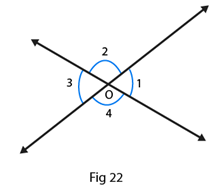Solution:

Given from the figure 22, ∠1 = ∠3 are the vertically opposite angles

Therefore, ∠3 = 65o

Here, ∠1 + ∠2 = 180° are the linear pair [The two adjacent angles are said to form a linear pair of angles if their non–common arms are two opposite rays and sum of the angle is 180o]

Therefore, ∠2 = 180o – 65o

= 115o

∠2 = ∠4 are the vertically opposite angles [from the figure]

Therefore, ∠2 = ∠4 = 115o

And ∠3 = 65o

22. In Fig. 23, OA and OB are opposite rays:

(i) If x = 25o, what is the value of y?

(ii) If y = 35o, what is the value of x?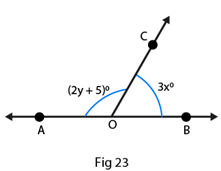Solution:

(i) ∠AOC + ∠BOC = 180o [The two adjacent angles are said to form a linear pair of angles if their non–common arms are two opposite rays and sum of the angle is 180o]

2y + 50 + 3x = 180o

3x + 2y = 175o

Given If x = 25o, then

3(25o) + 2y = 175o

75o + 2y = 175o

2y = 175o – 75o

2y = 100o

y = 100o/2

y = 50o

(ii) ∠AOC + ∠BOC = 180o [The two adjacent angles are said to form a linear pair of angles if their non–common arms are two opposite rays and sum of the angle is 180o]

2y + 5 + 3x = 180o

3x + 2y = 175o

Given If y = 35o, then

3x + 2(35o) = 175o

3x + 70o = 175o

3x = 1750 – 70o

3x = 105o

x = 105o/3

x = 35o

23. In Fig. 24, write all pairs of adjacent angles and all the liner pairs.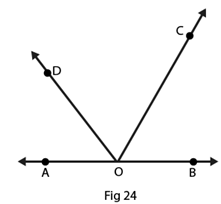Solution:

∠DOA and ∠DOC

∠BOC and ∠COD

∠AOD and ∠BOD

∠AOC and ∠BOC

Linear pairs: [The two adjacent angles are said to form a linear pair of angles if their non–common arms are two opposite rays and sum of the angle is 180o]

∠AOD and ∠BOD

∠AOC and ∠BOC

24. In Fig. 25, find ∠x. Further find ∠BOC, ∠COD and ∠AOD.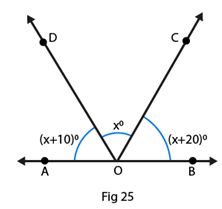Solution:

(x + 10)o + xo + (x + 20)o = 180o[linear pair]

On rearranging we get

3xo + 30o = 180o

3xo = 180o – 30o

3xo = 150o

xo = 150o/3

xo = 50o

Also given that

∠BOC = (x + 20)o

= (50 + 20)o

= 70o

∠COD = 50o

∠AOD = (x + 10)o

= (50 + 10)o

= 60o

25. How many pairs of adjacent angles are formed when two lines intersect in a point?

Solution:

If the two lines intersect at a point, then four adjacent pairs are formed and those are linear.

26. How many pairs of adjacent angles, in all, can you name in Fig. 26?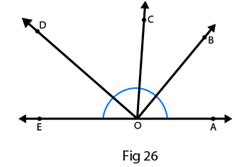Solution:

There are 10 adjacent pairs formed in the given figure, they are

∠EOD and ∠DOC

∠COD and ∠BOC

∠COB and ∠BOA

∠AOB and ∠BOD

∠BOC and ∠COE

∠COD and ∠COA

∠DOE and ∠DOB

∠EOD and ∠DOA

∠EOC and ∠AOC

∠AOB and ∠BOE

27. In Fig. 27, determine the value of x.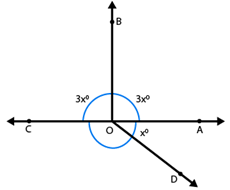Solution:

From the figure we can write as ∠COB + ∠AOB = 180o [linear pair]

3xo + 3xo = 180o

6xo = 180o

xo = 180o/6

xo = 30o

28. In Fig.28, AOC is a line, find x.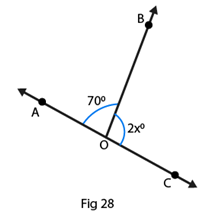Solution:

From the figure we can write as

∠AOB + ∠BOC = 180o [linear pair]

Linear pair

2x + 70o = 180o

2x = 180o – 70o

2x = 110o

x = 110o/2

x = 55o

29. In Fig. 29, POS is a line, find x.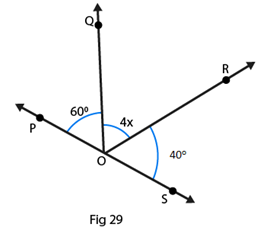Solution:

From the figure we can write as angles of a straight line,

∠QOP + ∠QOR + ∠ROS = 180o

60o + 4x + 40o = 180o

On rearranging we get, 100o + 4x = 180o

4x = 180o – 100o

4x = 80o

x = 80o/4

x = 20o

30. In Fig. 30, lines l1 and l2 intersect at O, forming angles as shown in the figure. If x = 45o, find the values of y, z and u.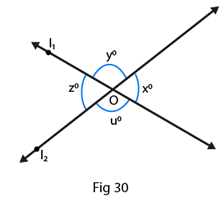Solution:

Given that, ∠x = 45o

From the figure we can write as

∠x = ∠z = 45o

Also from the figure, we have

∠y = ∠u

From the property of linear pair we can write as

∠x + ∠y + ∠z + ∠u = 360o

45o + 45o + ∠y + ∠u = 360o

90o + ∠y + ∠u = 360o

∠y + ∠u = 360o – 90o

∠y + ∠u = 270o (vertically opposite angles ∠y = ∠u)

2∠y = 270o

∠y = 135o

Therefore, ∠y = ∠u = 135o

So, ∠x = 45o, ∠y = 135o, ∠z = 45o and ∠u = 135o

31. In Fig. 31, three coplanar lines intersect at a point O, forming angles as shown in the figure. Find the values of x, y, z and u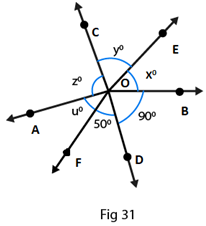Solution:

Given that, ∠x + ∠y + ∠z+ ∠u + 50o + 90o = 360o

Linear pair, ∠x + 50o + 90o = 180o

∠x + 140o = 180o

On rearranging we get

∠x = 180o – 140o

∠x = 40o

From the figure we can write as

∠x = ∠u = 40o are vertically opposite angles

∠z = 90o is a vertically opposite angle

∠y = 50o is a vertically opposite angle

Therefore, ∠x = 40o, ∠y = 50o, ∠z = 90o and ∠u = 40o

32. In Fig. 32, find the values of x, y and z.Solution:

∠y = 25o vertically opposite angle

From the figure we can write as

∠x = ∠z are vertically opposite angles

∠x + ∠y + ∠z + 25o = 360o

∠x + ∠z + 25o + 25o = 360o

On rearranging we get,

∠x + ∠z + 50o = 360o

∠x + ∠z = 360o – 50o [∠x = ∠z]

2∠x = 310o

∠x = 155o

And, ∠x = ∠z = 155o

Therefore, ∠x = 155o, ∠y = 25o and ∠z = 155o

Exercise 14.2 Page No: 14.20

1. In Fig. 58, line n is a transversal to line l and m. Identify the following:

(i) Alternate and corresponding angles in Fig. 58 (i)

(ii) Angles alternate to ∠d and ∠g and angles corresponding to ∠f and ∠h in Fig. 58 (ii)

(iii) Angle alternate to ∠PQR, angle corresponding to ∠RQF and angle alternate to ∠PQE in Fig. 58 (iii)

(iv) Pairs of interior and exterior angles on the same side of the transversal in Fig. 58 (ii)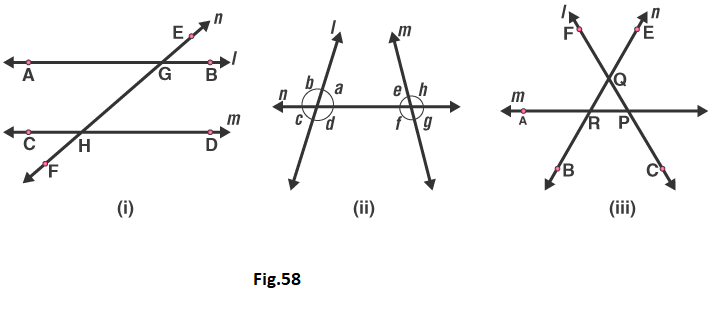Solution:

(i) A pair of angles in which one arm of both the angles is on the same side of the transversal and their other arms are directed in the same sense is called a pair of corresponding angles.

In Figure (i) Corresponding angles are

∠EGB and ∠GHD

∠HGB and ∠FHD

∠EGA and ∠GHC

∠AGH and ∠CHF

A pair of angles in which one arm of each of the angle is on opposite sides of the transversal and whose other arms include the one segment is called a pair of alternate angles.

The alternate angles are:

∠EGB and ∠CHF

∠HGB and ∠CHG

∠EGA and ∠FHD

∠AGH and ∠GHD

(ii) In Figure (ii)

The alternate angle to ∠d is ∠e.

The alternate angle to ∠g is ∠b.

The corresponding angle to ∠f is ∠c.

The corresponding angle to ∠h is ∠a.

(iii) In Figure (iii)

Angle alternate to ∠PQR is ∠QRA.

Angle corresponding to ∠RQF is ∠ARB.

Angle alternate to ∠POE is ∠ARB.

(iv) In Figure (ii)

Pair of interior angles are

∠a is ∠e.

∠d is ∠f.

Pair of exterior angles are

∠b is ∠h.

∠c is ∠g.

2. In Fig. 59, AB and CD are parallel lines intersected by a transversal PQ at L and M respectively, If ∠CMQ = 60o, find all other angles in the figure.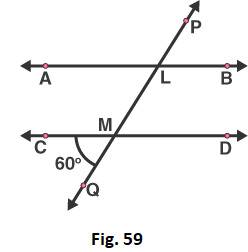Solution:

A pair of angles in which one arm of both the angles is on the same side of the transversal and their other arms are directed in the same sense is called a pair of corresponding angles.

Therefore corresponding angles are

∠ALM = ∠CMQ = 60o [given]

Vertically opposite angles are

∠LMD = ∠CMQ = 60o [given]

Vertically opposite angles are

∠ALM = ∠PLB = 60o

Here, ∠CMQ + ∠QMD = 180o are the linear pair

On rearranging we get

∠QMD = 180o – 60o

= 120o

Corresponding angles are

∠QMD = ∠MLB = 120o

Vertically opposite angles

∠QMD = ∠CML = 120o

Vertically opposite angles

∠MLB = ∠ALP = 120o

3. In Fig. 60, AB and CD are parallel lines intersected by a transversal by a transversal PQ at L and M respectively. If ∠LMD = 35o find ∠ALM and ∠PLA.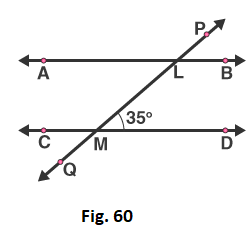Solution:

Given that, ∠LMD = 35o

From the figure we can write

∠LMD and ∠LMC is a linear pair

∠LMD + ∠LMC = 180o [sum of angles in linear pair = 180o]

On rearranging, we get

∠LMC = 180o – 35o

= 145o

So, ∠LMC = ∠PLA = 145o

And, ∠LMC = ∠MLB = 145o

∠MLB and ∠ALM is a linear pair

∠MLB + ∠ALM = 180o [sum of angles in linear pair = 180o]

∠ALM = 180o – 145o

∠ALM = 350

Therefore, ∠ALM = 35o, ∠PLA = 145o.

4. The line n is transversal to line l and m in Fig. 61. Identify the angle alternate to ∠13, angle corresponding to ∠15, and angle alternate to ∠15.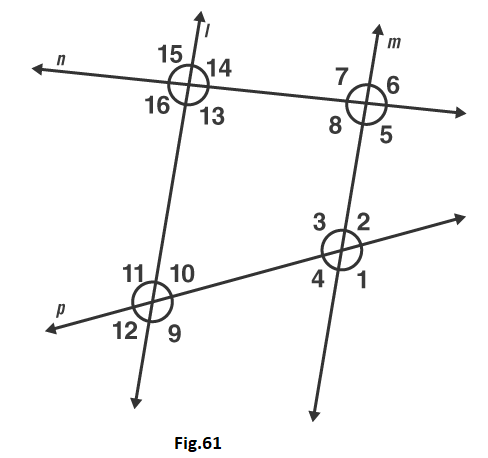Solution:

Given that, l ∥ m

From the figure the angle alternate to ∠13 is ∠7

From the figure the angle corresponding to ∠15 is ∠7 [A pair of angles in which one arm of both the angles is on the same side of the transversal and their other arms are directed in the same sense is called a pair of corresponding angles.]

Again from the figure angle alternate to ∠15 is ∠5

5. In Fig. 62, line l ∥ m and n is transversal. If ∠1 = 40°, find all the angles and check that all corresponding angles and alternate angles are equal.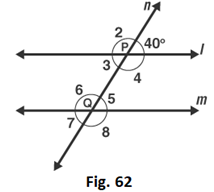Solution:

Given that, ∠1 = 40o

∠1 and ∠2 is a linear pair [from the figure]

∠1 + ∠2 = 180o

∠2 = 180o – 40o

∠2 = 140o

Again from the figure we can say that

∠2 and ∠6 is a corresponding angle pair

So, ∠6 = 140o

∠6 and ∠5 is a linear pair [from the figure]

∠6 + ∠5 = 180o

∠5 = 180o – 140o

∠5 = 40o

From the figure we can write as

∠3 and ∠5 are alternate interior angles

So, ∠5 = ∠3 = 40o

∠3 and ∠4 is a linear pair

∠3 + ∠4 = 180o

∠4 = 180o – 40o

∠4 = 140o

Now, ∠4 and ∠6 are a pair of interior angles

So, ∠4 = ∠6 = 140o

∠3 and ∠7 are a pair of corresponding angles

So, ∠3 = ∠7 = 40o

Therefore, ∠7 = 40o

∠4 and ∠8 are a pair of corresponding angles

So, ∠4 = ∠8 = 140o

Therefore, ∠8 = 140o

Therefore, ∠1 = 40o, ∠2 = 140o, ∠3 = 40o, ∠4 = 140o, ∠5 = 40o, ∠6 = 140o, ∠7 = 40o and ∠8 = 140o

6. In Fig.63, line l ∥ m and a transversal n cuts them P and Q respectively. If ∠1 = 75°, find all other angles.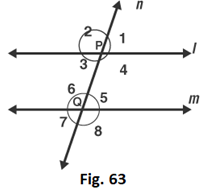Solution:

Given that, l ∥ m and ∠1 = 75o

∠1 = ∠3 are vertically opposite angles

We know that, from the figure

∠1 + ∠2 = 180o is a linear pair

∠2 = 180o – 75o

∠2 = 105o

Here, ∠1 = ∠5 = 75o are corresponding angles

∠5 = ∠7 = 75o are vertically opposite angles.

∠2 = ∠6 = 105o are corresponding angles

∠6 = ∠8 = 105o are vertically opposite angles

∠2 = ∠4 = 105o are vertically opposite angles

So, ∠1 = 75o, ∠2 = 105o, ∠3 = 75o, ∠4 = 105o, ∠5 = 75o, ∠6 = 105o, ∠7 = 75o and ∠8 = 105o

7. In Fig. 64, AB ∥ CD and a transversal PQ cuts at L and M respectively. If ∠QMD = 100o, find all the other angles.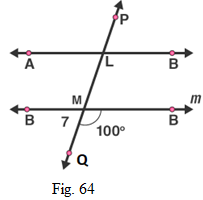Solution:

Given that, AB ∥ CD and ∠QMD = 100o

We know that, from the figure ∠QMD + ∠QMC = 180o is a linear pair,

∠QMC = 180o – ∠QMD

∠QMC = 180o – 100°

∠QMC = 80o

Corresponding angles are

∠DMQ = ∠BLM = 100o

∠CMQ = ∠ALM = 80o

Vertically Opposite angles are

∠DMQ = ∠CML = 100o

∠BLM = ∠PLA = 100o

∠CMQ = ∠DML = 80o

∠ALM = ∠PLB = 80o

8. In Fig. 65, l ∥ m and p ∥ q. Find the values of x, y, z, t.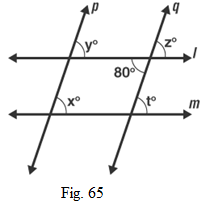Solution:

Given that one of the angle is 80o

∠z and 80o are vertically opposite angles

Therefore ∠z = 80o

∠z and ∠t are corresponding angles

∠z = ∠t

Therefore, ∠t = 80o

∠z and ∠y are corresponding angles

∠z = ∠y

Therefore, ∠y = 80o

∠x and ∠y are corresponding angles

∠y = ∠x

Therefore, ∠x = 80o

9. In Fig. 66, line l ∥ m, ∠1 = 120o and ∠2 = 100o, find out ∠3 and ∠4.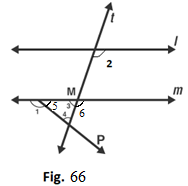Solution:

Given that, ∠1 = 120o and ∠2 = 100o

From the figure ∠1 and ∠5 is a linear pair

∠1 + ∠5 = 180o

∠5 = 180o – 120o

∠5 = 60o

Therefore, ∠5 = 60o

∠2 and ∠6 are corresponding angles

∠2 = ∠6 = 100o

Therefore, ∠6 = 100o

∠6 and ∠3 a linear pair

∠6 + ∠3 = 180o

∠3 = 180o – 100o

∠3 = 80o

Therefore, ∠3 = 80o

By, angles of sum property

∠3 + ∠5 + ∠4 = 180o

∠4 = 180o – 80o – 60o

∠4 = 40o

Therefore, ∠4 = 40o

10.  In Fig. 67, l ∥ m. Find the values of a, b, c, d. Give reasons.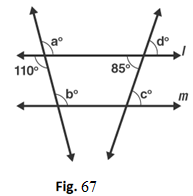Solution:

Given l ∥ m

From the figure vertically opposite angles,

∠a = 110o

Corresponding angles, ∠a = ∠b

Therefore, ∠b = 110o

Vertically opposite angle,

∠d = 85o

Corresponding angles, ∠d = ∠c

Therefore, ∠c = 85o

Hence, ∠a = 110o, ∠b = 110o, ∠c = 85o, ∠d = 85o

11. In Fig. 68, AB ∥ CD and ∠1 and ∠2 are in the ratio of 3: 2. Determine all angles from 1 to 8.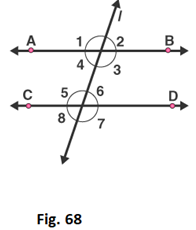Solution:

Given ∠1 and ∠2 are in the ratio 3: 2

Let us take the angles as 3x, 2x

∠1 and ∠2 are linear pair [from the figure]

3x + 2x = 180o

5x = 180o

x = 180o/5

x = 36o

Therefore, ∠1 = 3x = 3(36) = 108o

∠2 = 2x = 2(36) = 72o

∠1 and ∠5 are corresponding angles

Therefore ∠1 = ∠5

Hence, ∠5 = 108o

∠2 and ∠6 are corresponding angles

So ∠2 = ∠6

Therefore, ∠6 = 72o

∠4 and ∠6 are alternate pair of angles

∠4 = ∠6 = 72o

Therefore, ∠4 = 72o

∠3 and ∠5 are alternate pair of angles

∠3 = ∠5 = 108o

Therefore, ∠3 = 108o

∠2 and ∠8 are alternate exterior of angles

∠2 = ∠8 = 72o

Therefore, ∠8 = 72o

∠1 and ∠7 are alternate exterior of angles

∠1 = ∠7 = 108o

Therefore, ∠7 = 108o

Hence, ∠1 = 108o, ∠2 = 72o, ∠3 = 108o, ∠4 = 72o, ∠5 = 108o, ∠6 = 72o, ∠7 = 108o, ∠8 = 72o

12. In Fig. 69, l, m and n are parallel lines intersected by transversal p at X, Y and Z respectively. Find ∠1, ∠2 and ∠3.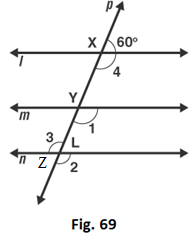Solution:

Given l, m and n are parallel lines intersected by transversal p at X, Y and Z

Therefore linear pair,

∠4 + 60o = 180o

∠4 = 180o – 60o

∠4 = 120o

From the figure,

∠4 and ∠1 are corresponding angles

∠4 = ∠1

Therefore, ∠1 = 120o

∠1 and ∠2 are corresponding angles

∠2 = ∠1

Therefore, ∠2 = 120o

∠2 and ∠3 are vertically opposite angles

∠2 = ∠3

Therefore, ∠3 = 1200

13. In Fig. 70, if l ∥ m ∥ n and ∠1 = 60o, find ∠2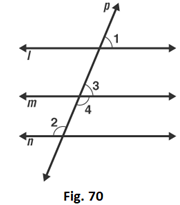Solution:

Given that l ∥ m ∥ n

From the figure Corresponding angles are

∠1 = ∠3

∠1 = 60o

Therefore, ∠3 = 60o

∠3 and ∠4 are linear pair

∠3 + ∠4 = 180o

∠4 = 180o – 60o

∠4 = 120o

∠2 and ∠4 are alternate interior angles

∠4 = ∠2

Therefore, ∠2 = 120o

14. In Fig. 71, if AB ∥ CD and CD ∥ EF, find ∠ACE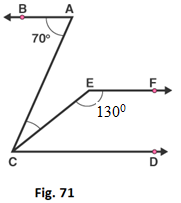Solution:

Given that, AB ∥ CD and CD ∥ EF

Sum of the interior angles,

∠CEF + ∠ECD = 180o

130o + ∠ECD = 180o

∠ECD = 180o – 130o

∠ECD = 50o

We know that alternate angles are equal

∠BAC = ∠ACD

∠BAC = ∠ECD + ∠ACE

∠ACE = 70o – 50o

∠ACE = 20o

Therefore, ∠ACE = 20o

15. In Fig. 72, if l ∥ m, n ∥ p and ∠1 = 85o, find ∠2.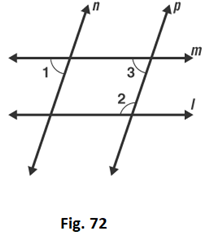Solution:

Given that, ∠1 = 85o

∠1 and ∠3 are corresponding angles

So, ∠1 = ∠3

∠3 = 85o

Sum of the interior angles is 180o

∠3 + ∠2 = 180o

∠2 = 180o – 85o

∠2 = 95o

16. In Fig. 73, a transversal n cuts two lines l and m. If ∠1 = 70o and ∠7 = 80o, is l ∥ m?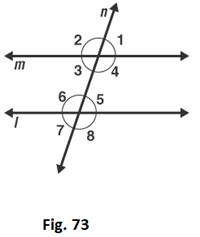Solution:

Given ∠1 = 70o and ∠7 = 80o

We know that if the alternate exterior angles of the two lines are equal, then the lines are parallel.

Here, ∠1 and ∠7 are alternate exterior angles, but they are not equal

∠1 ≠ ∠7

17. In Fig. 74, a transversal n cuts two lines l and m such that ∠2 = 65o and ∠8 = 65o. Are the lines parallel?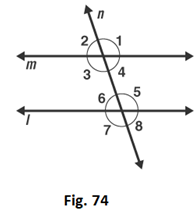Solution:

From the figure ∠2 = ∠4 are vertically opposite angles,

∠2 = ∠4 = 65o

∠8 = ∠6 = 65o

Therefore, ∠4 = ∠6

Hence, l ∥ m

18. In Fig. 75, Show that AB ∥ EF.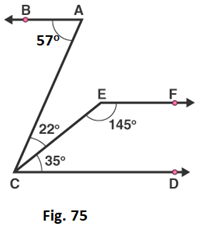Solution:

We know that,

∠ACD = ∠ACE + ∠ECD

∠ACD = 22o + 35o

∠ACD = 57o = ∠BAC

Thus, lines BA and CD are intersected by the line AC such that, ∠ACD = ∠BAC

So, the alternate angles are equal

Therefore, AB ∥ CD ……1

Now,

∠ECD + ∠CEF = 35o + 145o = 180o

This, shows that sum of the angles of the interior angles on the same side of the transversal CE is 180o

So, they are supplementary angles

Therefore, EF ∥ CD …….2

From equation 1 and 2

We conclude that, AB ∥ EF

19. In Fig. 76, AB ∥ CD. Find the values of x, y, z.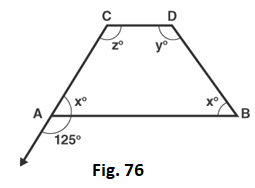Solution:

Given that AB ∥ CD

Linear pair,

∠x + 125o = 180o

∠x = 180o – 125o

∠x = 55o

Corresponding angles

∠z = 125o

∠x + ∠z = 180o

∠x + 125o = 180o

∠x = 180o – 125o

∠x = 55o

∠x + ∠y = 180o

∠y + 55o = 180o

∠y = 180o – 55o

∠y = 125o

20. In Fig. 77, find out ∠PXR, if PQ ∥ RS.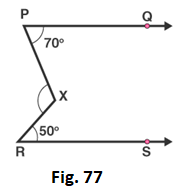Solution:

Given PQ ∥ RS

We need to find ∠PXR

∠XRS = 50o

∠XPQ = 70o

Given, that PQ ∥ RS

∠PXR = ∠XRS + ∠XPR

∠PXR = 50o + 70o

∠PXR = 120o

Therefore, ∠PXR = 120o

21. In Figure, we have

(i) ∠MLY = 2∠LMQ

(ii) ∠XLM = (2x – 10)o and ∠LMQ = (x + 30)o, find x.

(iii) ∠XLM = ∠PML, find ∠ALY

(iv) ∠ALY = (2x – 15)o, ∠LMQ = (x + 40)o , find x.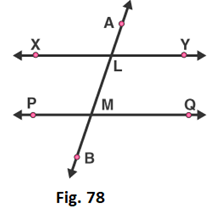Solution:

(i) ∠MLY and ∠LMQ are interior angles

∠MLY + ∠LMQ = 180o

2∠LMQ + ∠LMQ = 180o

3∠LMQ = 180o

∠LMQ = 180o/3

∠LMQ = 60o

(ii) ∠XLM = (2x – 10)o and ∠LMQ = (x + 30)o, find x.

∠XLM = (2x – 10)o and ∠LMQ = (x + 30)o

∠XLM and ∠LMQ are alternate interior angles

∠XLM = ∠LMQ

(2x – 10)o = (x + 30)o

2x – x = 30o + 10o

x = 40o

Therefore, x = 40°

(iii) ∠XLM = ∠PML, find ∠ALY

∠XLM = ∠PML

Sum of interior angles is 180 degrees

∠XLM + ∠PML = 180o

∠XLM + ∠XLM = 180o

2∠XLM = 180o

∠XLM = 180o/2

∠XLM = 90o

∠XLM and ∠ALY are vertically opposite angles

Therefore, ∠ALY = 90o

(iv) ∠ALY = (2x – 15)o, ∠LMQ = (x + 40)o, find x.

∠ALY and ∠LMQ are corresponding angles

∠ALY = ∠LMQ

(2x – 15)o = (x + 40)o

2x – x = 40o + 15o

x = 55o

Therefore, x = 55o

22. In Fig. 79, DE ∥ BC. Find the values of x and y.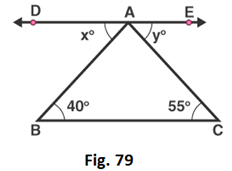Solution:

We know that,

ABC, DAB are alternate interior angles

∠ABC = ∠DAB

So, x = 40o

And ACB, EAC are alternate interior angles

∠ACB = ∠EAC

So, y = 55o

23. In Fig. 80, line AC ∥ line DE and ∠ABD = 32o, Find out the angles x and y if ∠E = 122o.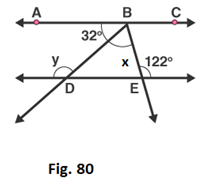Solution:

Given line AC ∥ line DE and ∠ABD = 32o

∠BDE = ∠ABD = 32o – Alternate interior angles

∠BDE + y = 180o– linear pair

32o + y = 180o

y = 180o – 32o

y = 148o

∠ABE = ∠E = 122o – Alternate interior angles

∠ABD + ∠DBE = 122o

32o + x = 122o

x = 122o – 32o

x = 90o

24. In Fig. 81, side BC of ΔABC has been produced to D and CE ∥ BA. If ∠ABC = 65o, ∠BAC = 55o, find ∠ACE, ∠ECD, ∠ACD.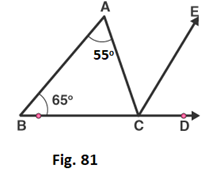Solution:

Given ∠ABC = 65o, ∠BAC = 55o

Corresponding angles,

∠ABC = ∠ECD = 65o

Alternate interior angles,

∠BAC = ∠ACE = 55o

Now, ∠ACD = ∠ACE + ∠ECD

∠ACD = 55o + 65o

= 120o

25. In Fig. 82, line CA ⊥ AB ∥ line CR and line PR ∥ line BD. Find ∠x, ∠y, ∠z.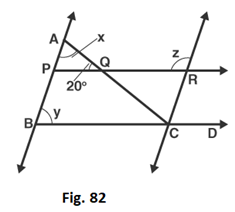Solution:

Given that, CA ⊥ AB

∠CAB = 90o

∠AQP = 20o

By, angle of sum property

In ΔABC

∠CAB + ∠AQP + ∠APQ = 180o

∠APQ = 180o – 90o – 20o

∠APQ = 70o

y and ∠APQ are corresponding angles

y = ∠APQ = 70o

∠APQ and ∠z are interior angles

∠APQ + ∠z = 180o

∠z = 180o – 70o

∠z = 110o

26. In Fig. 83, PQ ∥ RS. Find the value of x.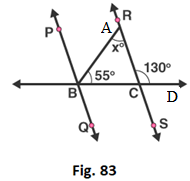Solution:

Given, linear pair,

∠RCD + ∠RCB = 180o

∠RCB = 180o – 130o

= 50o

In ΔABC,

∠BAC + ∠ABC + ∠BCA = 180o

By, angle sum property

∠BAC = 180o – 55o – 50o

∠BAC = 75o

27. In Fig. 84, AB ∥ CD and AE ∥ CF, ∠FCG = 90o and ∠BAC = 120o. Find the value of x, y and z.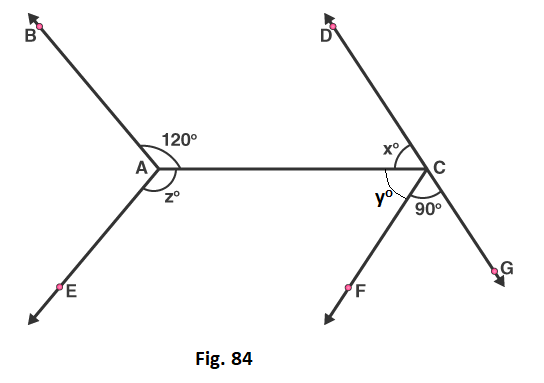Solution:

Alternate interior angle

∠BAC = ∠ACG = 120o

∠ACF + ∠FCG = 120o

So, ∠ACF = 120o – 90o

= 30o

Linear pair,

∠DCA + ∠ACG = 180o

∠x = 180o – 120o

= 60o

∠BAC + ∠BAE + ∠EAC = 360o

∠CAE = 360o – 120o – (60o + 30o)

= 150o

28. In Fig. 85, AB ∥ CD and AC ∥ BD. Find the values of x, y, z.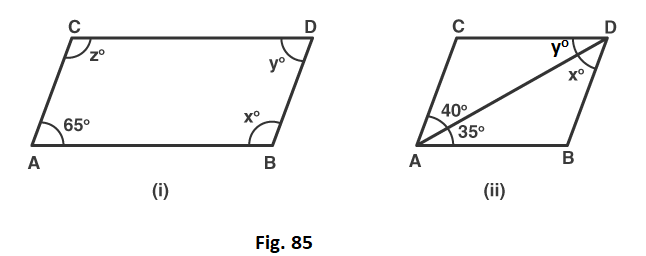Solution:

(i)  Since, AC ∥ BD and CD ∥ AB, ABCD is a parallelogram

∠CAB + ∠ACD = 180o

∠ACD = 180o – 65o

= 115o

Opposite angles of parallelogram,

∠CAB = ∠CDB = 65o

∠ACD = ∠DBA = 115o

(ii)  Here,

AC ∥ BD and CD ∥ AB

Alternate interior angles,

∠DAB = y = 35o

29. In Fig. 86, state which lines are parallel and why?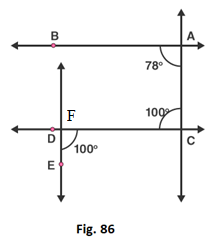Solution:

Let, F be the point of intersection of the line CD and the line passing through point E.

Here, ∠ACD and ∠CDE are alternate and equal angles.

So, ∠ACD = ∠CDE = 100o

Therefore, AC ∥ EF

30. In Fig. 87, the corresponding arms of ∠ABC and ∠DEF are parallel. If ∠ABC = 75o, find ∠DEF.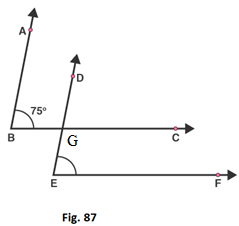Solution:

Let, G be the point of intersection of the lines BC and DE

Since, AB ∥ DE and BC ∥ EF

The corresponding angles are,

∠ABC = ∠DGC = ∠DEF = 75o